# Use Fibonacci tools

## Use Fibonacci toolsOn MetaTrader4 (MT4) / MetaTrader5 (MT5), you can use the technical indicators based on the Fibonacci sequence invented by Leonardo Fibonacci (Italy): "Fibonacci retracement", "Fibonacci time zones", "Fibonacci fan", "Fibonacci arcs", "Fibonacci channel", and "Fibonacci expansion".

Here we'll take a look at how to display and set up the simplest Fibonacci tool, the Fibonacci retracement.

Switch between MT4/MT5 tabs to check the steps for each.## Step 1

Fibonacci tools are available from the menu or the Toolbar.

### Draw Fibonacci retracement from the menu

Click "Insert" in the menu. Hover the pointer over "Fibonacci" and select "Retracement".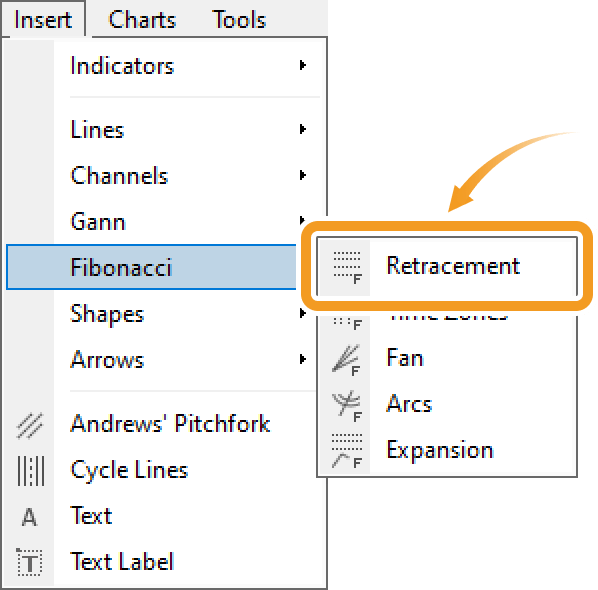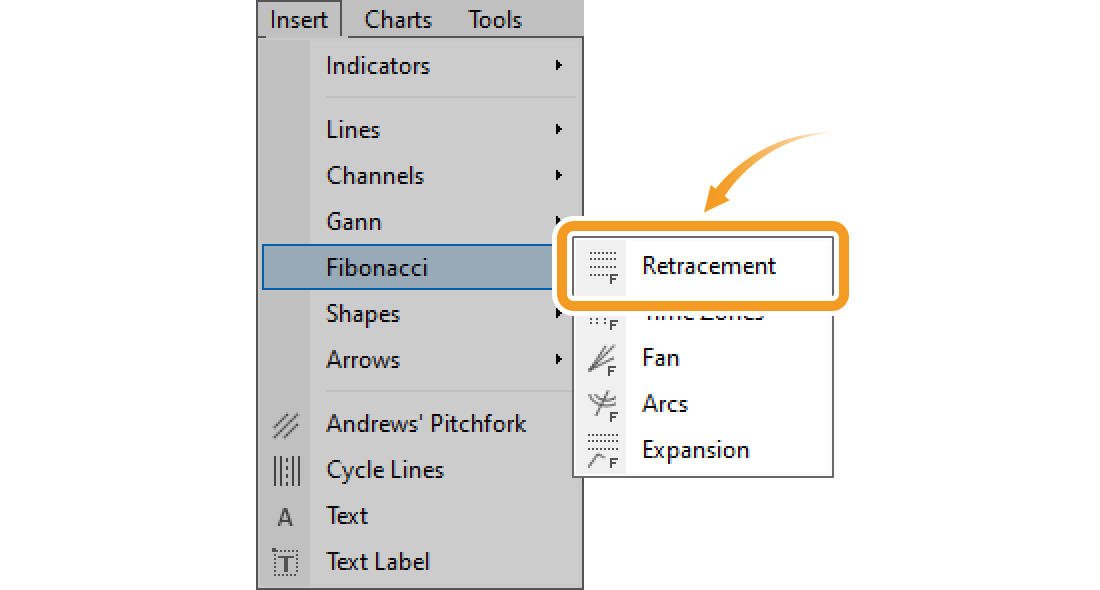### Draw Fibonacci retracement from the toolbar

Click the "Draw Fibonacci retracement" button in the toolbar.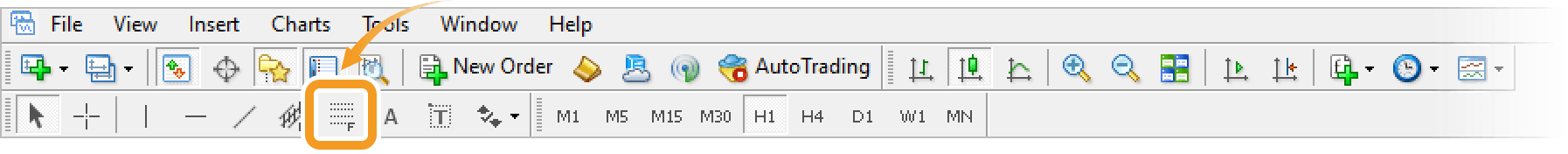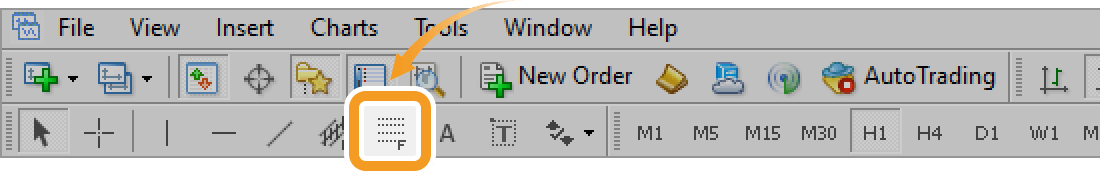1. Fibonacci retracement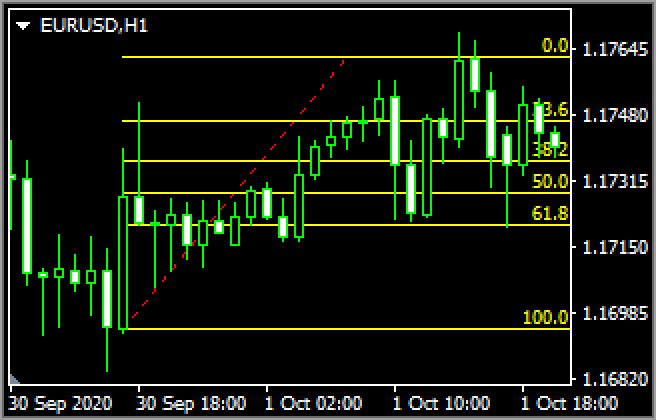Fibonacci retracement is a technical indicator that determines the temporary retrace price. It uses the Fibonacci ratio on the gap between the high and low to determine the dip/rally.

2. Fibonacci time zones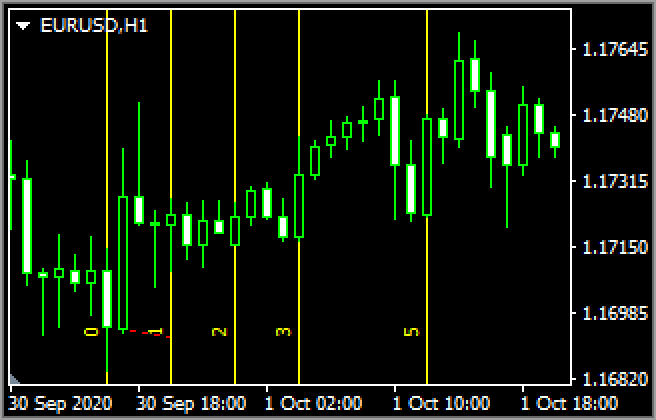Fibonacci time zones draw vertical Fibonacci lines relative to the time axis. Use these as reference points to determine when the trend will change.

3. Fibonacci fan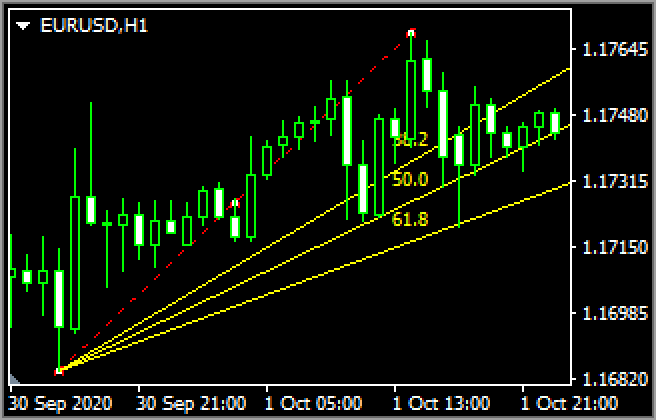Fibonacci fan draws 3 separate trendlines distanced from a starting point based on the Fibonacci ratio when you select the starting and end points of a wave. Use these lines as reference points to determine the support line and resistance line.

1. Fibonacci arcs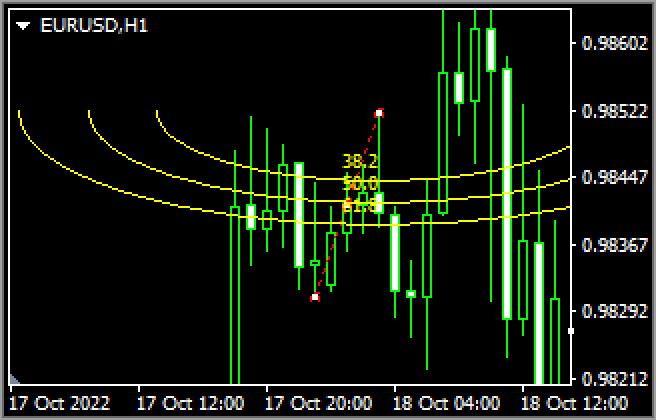Fibonacci arc is a technical indicator that utilizes the Fibonacci ratio on the price gap and time. Connect the high and low and it will draw 3 arcs relative to the end point using the Fibonacci ratio. Use these lines as reference points to determine the support line and resistance line.

2. Fibonacci expansion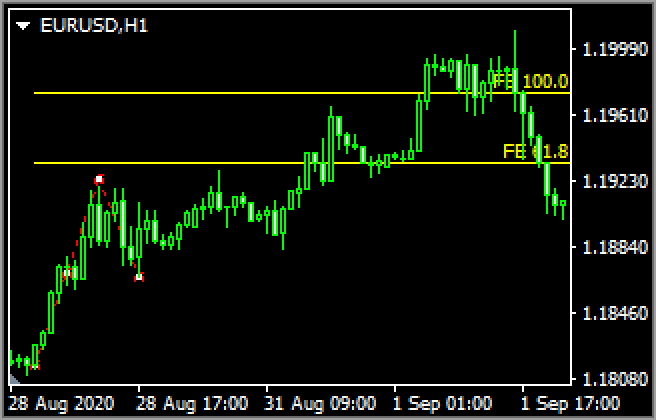Fibonacci expansion is a technical indicator that determines how long a trend will last. Connect the starting point of the trend and the dip/rally points and it will draw horizontal lines using the Fibonacci ratio. Use these as reference points to determine how far the market will expand.Fibonacci channel is under "Channels"

On MT5, you can find the Fibonacci channel in the "Fibonacci" menu, while on MT4, it is in the "Channels" menu.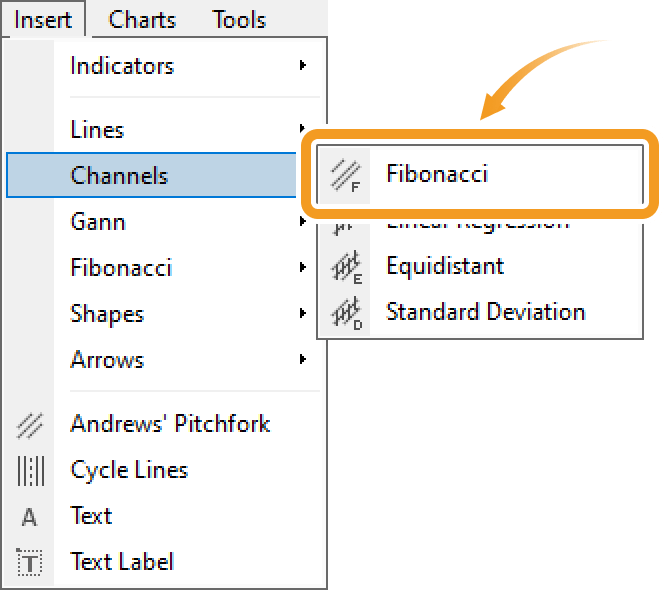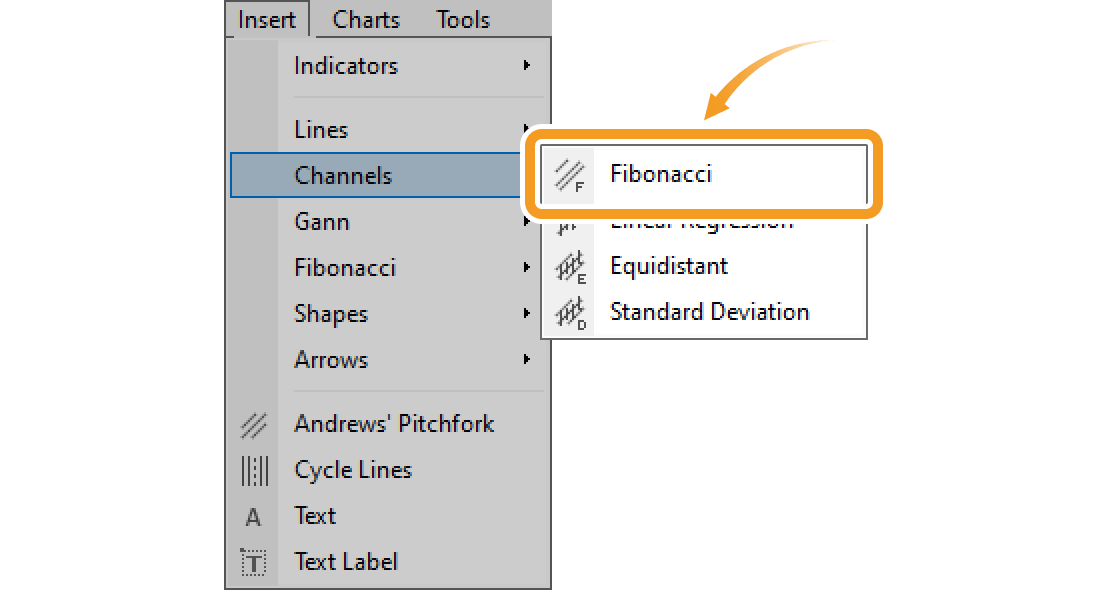## Step 2

Click the starting point on the chart and drag it to the end point to create the Fibonacci retracement.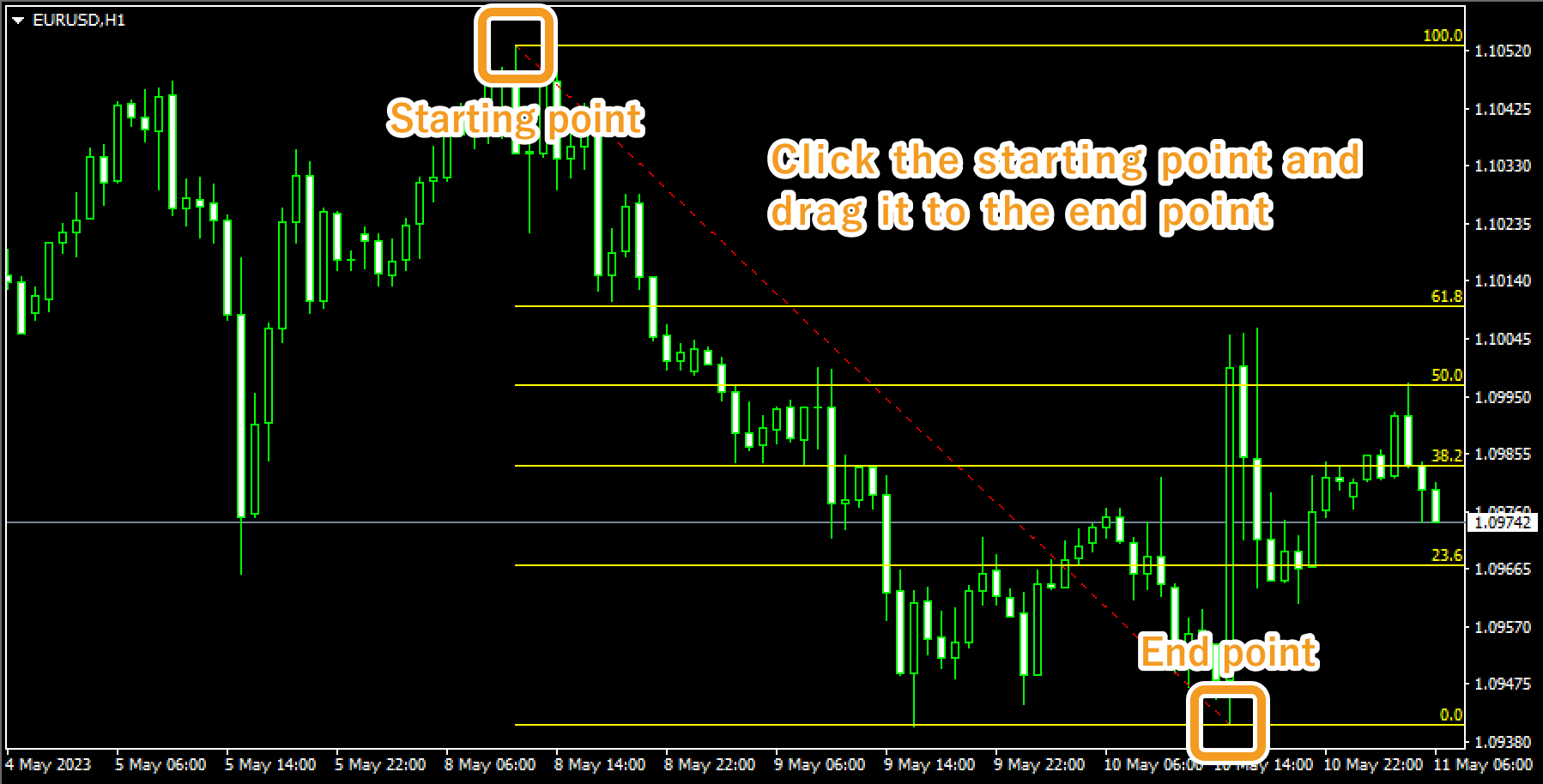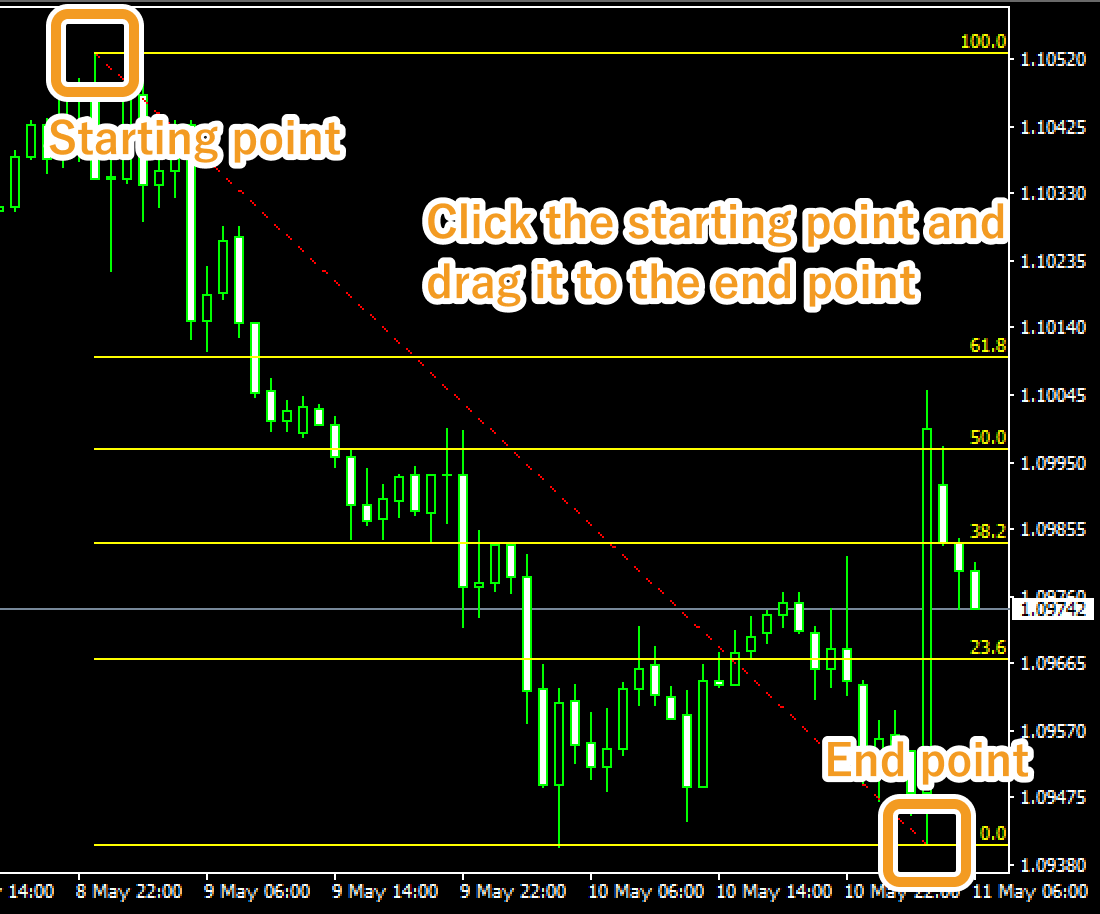## Step 3

If you want to adjust the position of the Fibonacci retracement, make sure the white dots are being displayed on the Fibonacci retracement and move each dot as necessary. If you cannot see the white dots, double-click near the line connecting the starting and end points to display the white dots.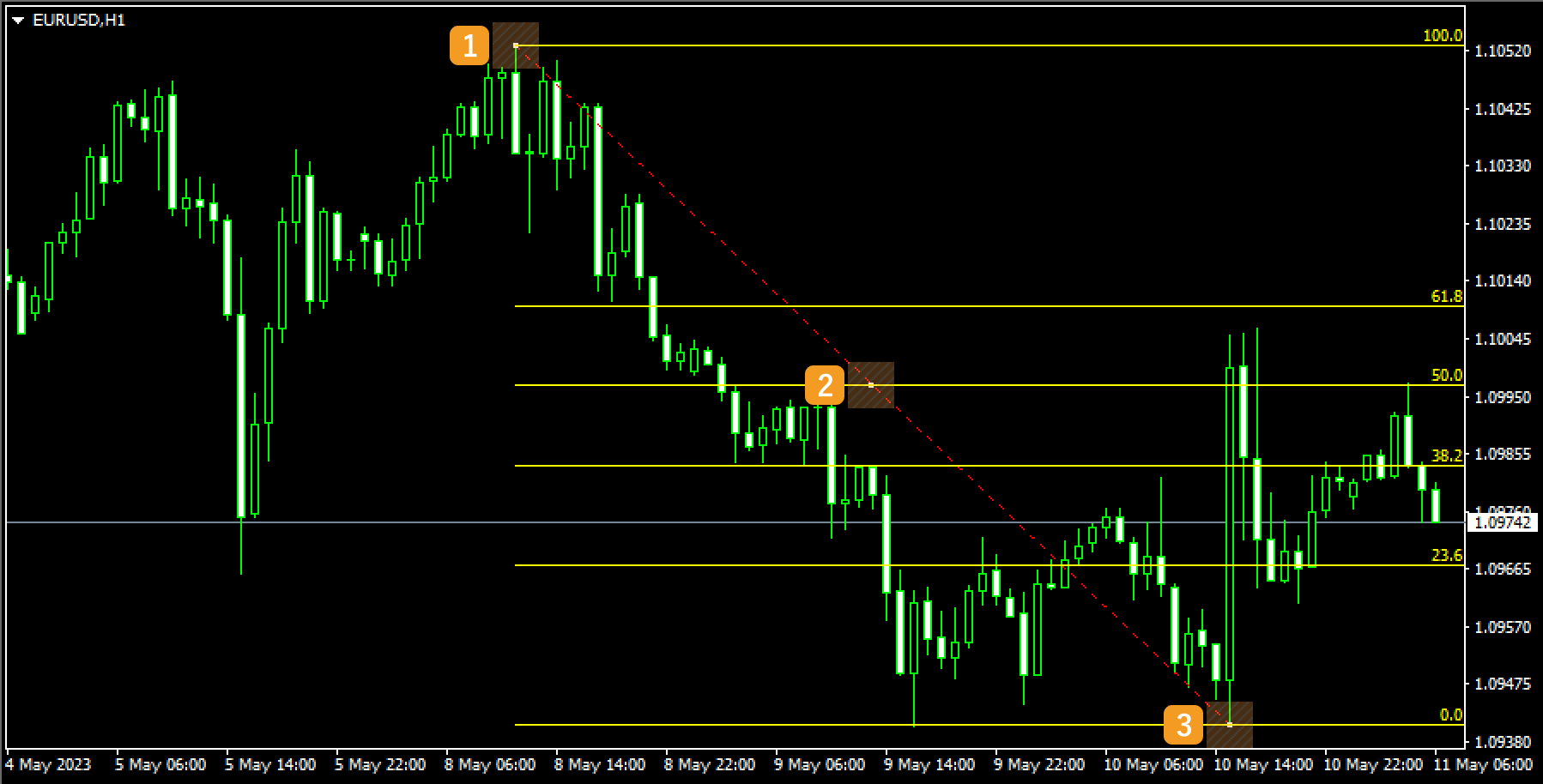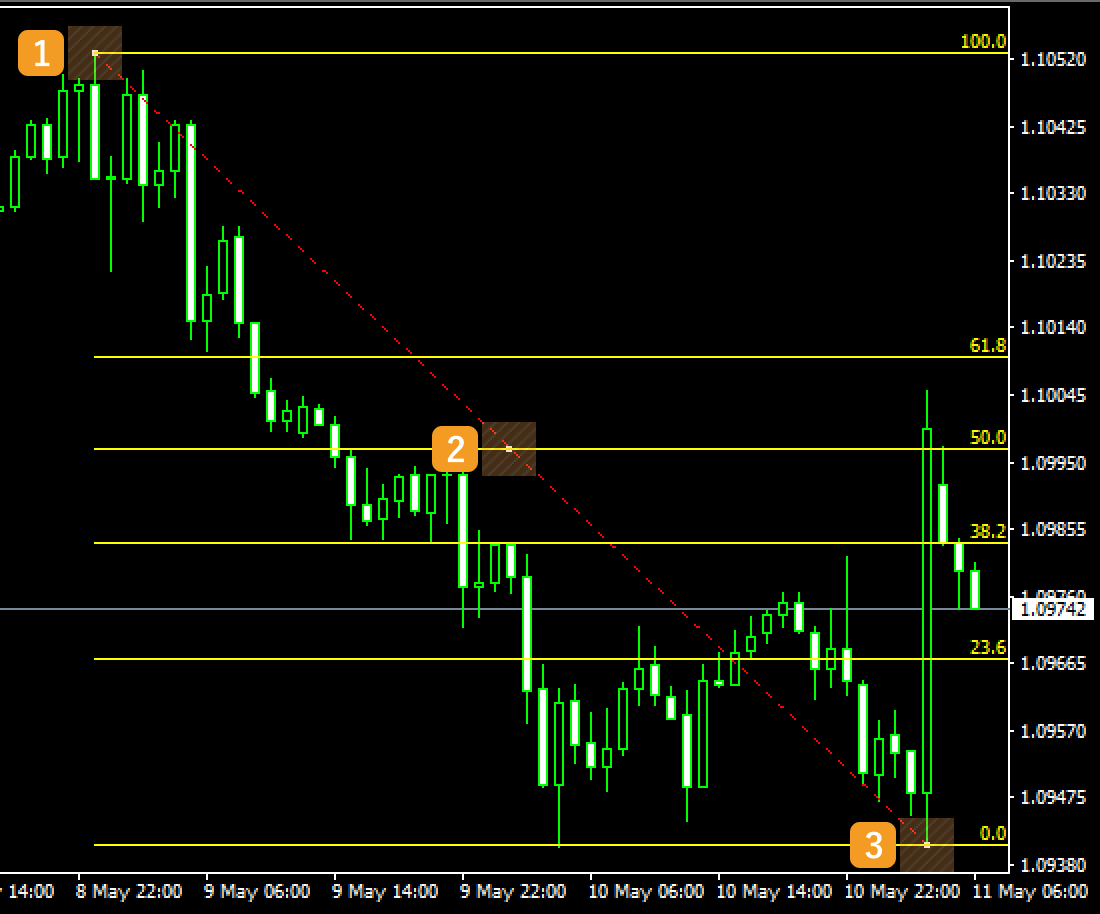Number Descriptions Change the starting point of the Fibonacci retracement. Change the position of the Fibonacci retracement. Change the end point of the Fibonacci retracement.Values displayed during editing Fibonacci retracement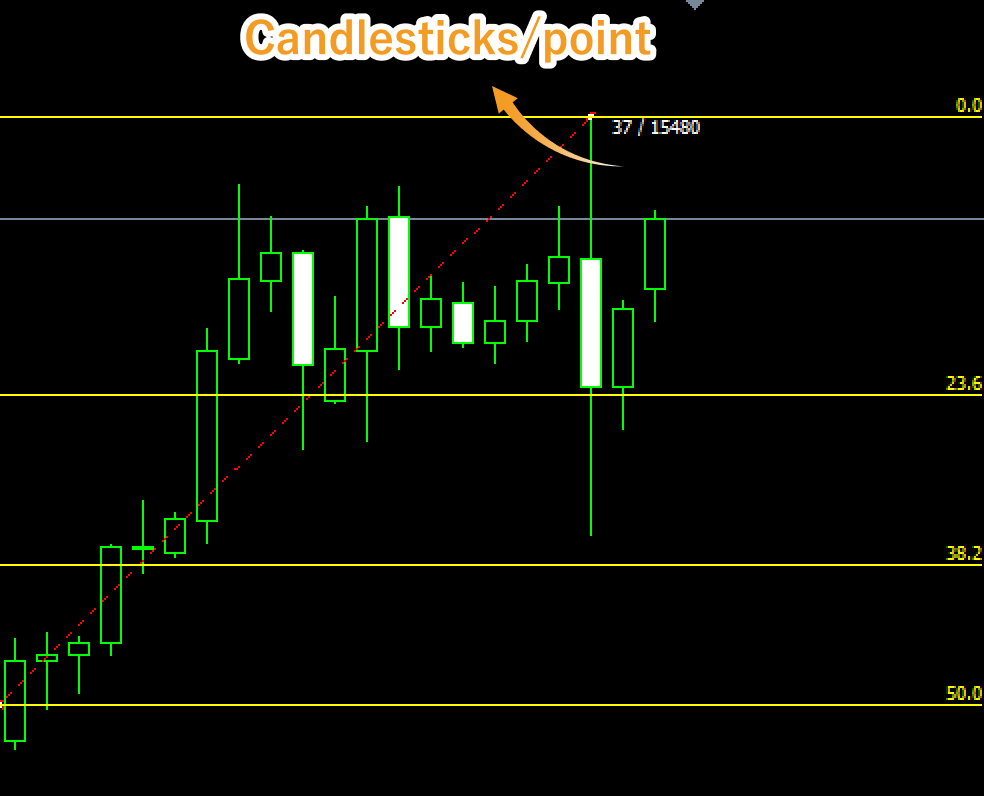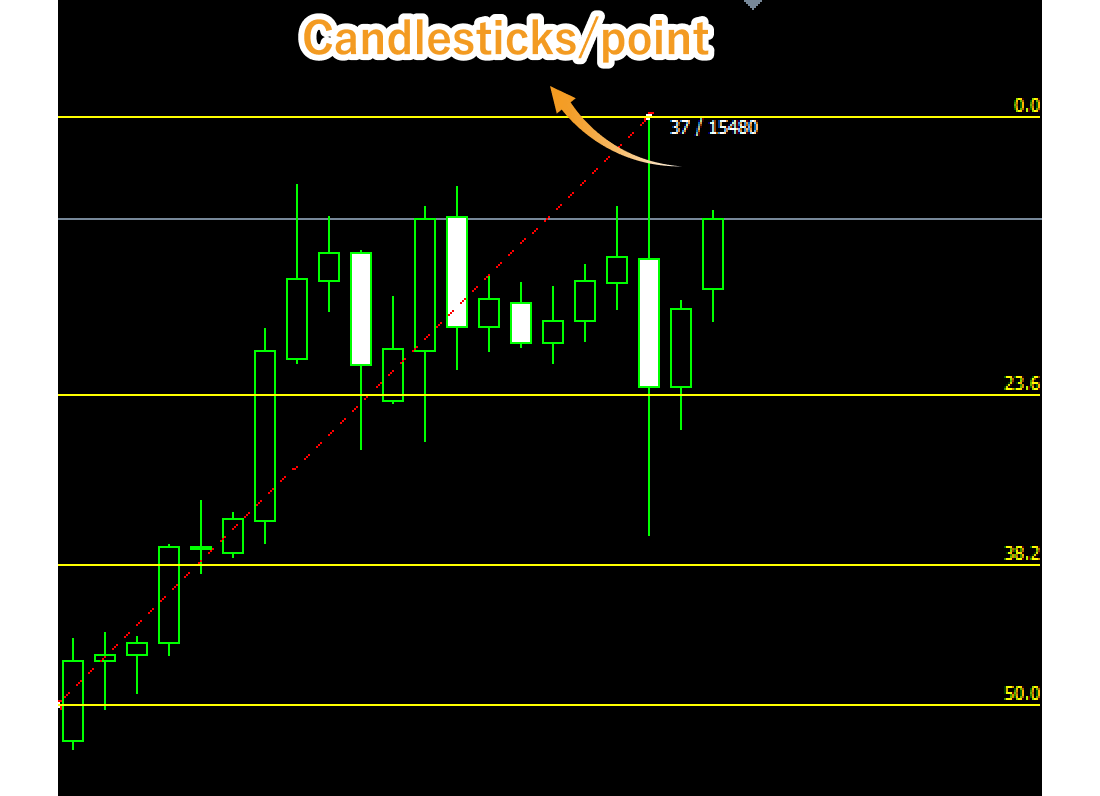Values displayed when dragging the starting or end point indicate the numbers of candlesticks and points from the starting point to the end point.

## Step 4

To modify the Fibonacci retracement settings, right-click on the line connecting the starting and end points and select "Fibo properties...".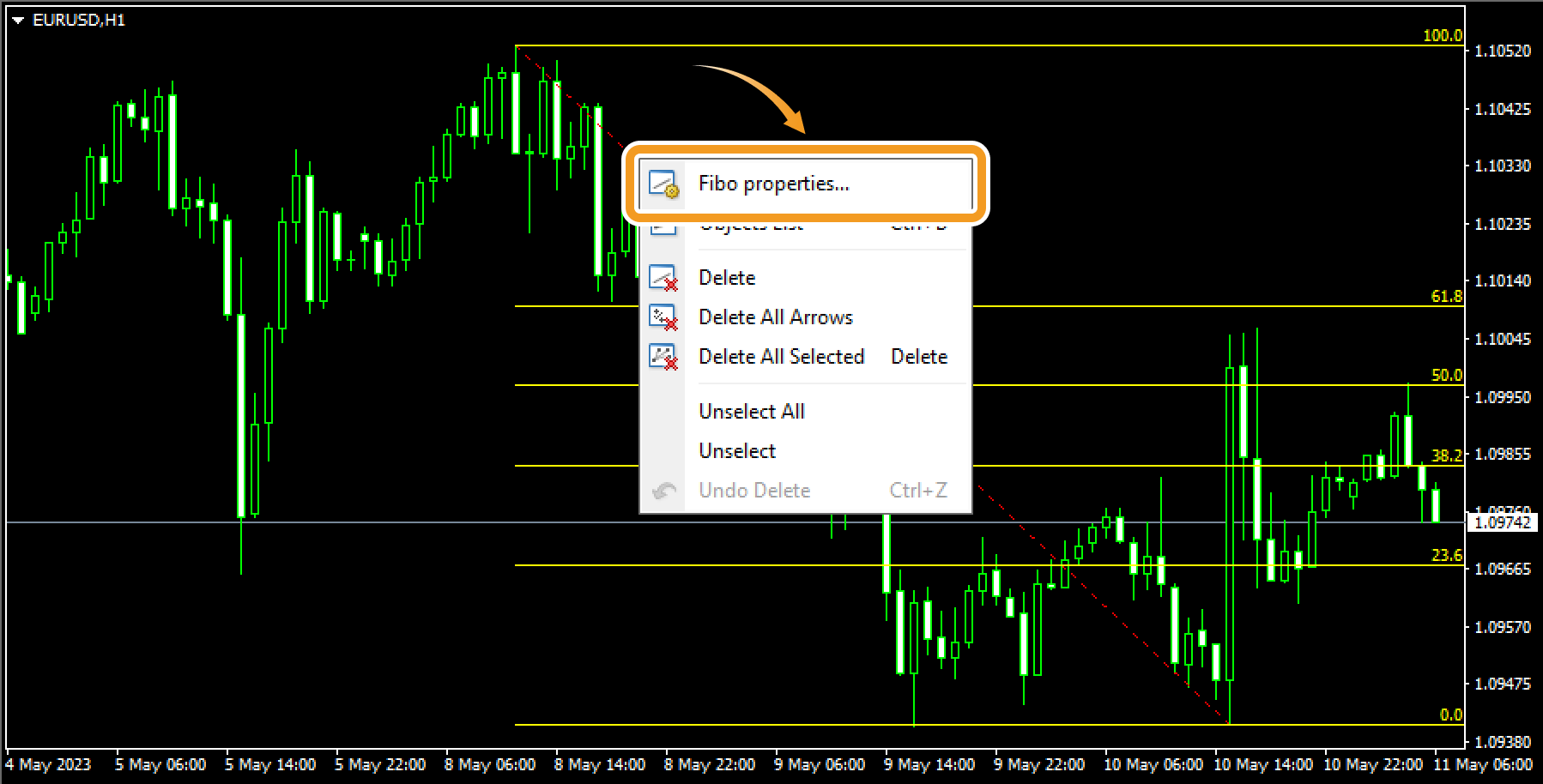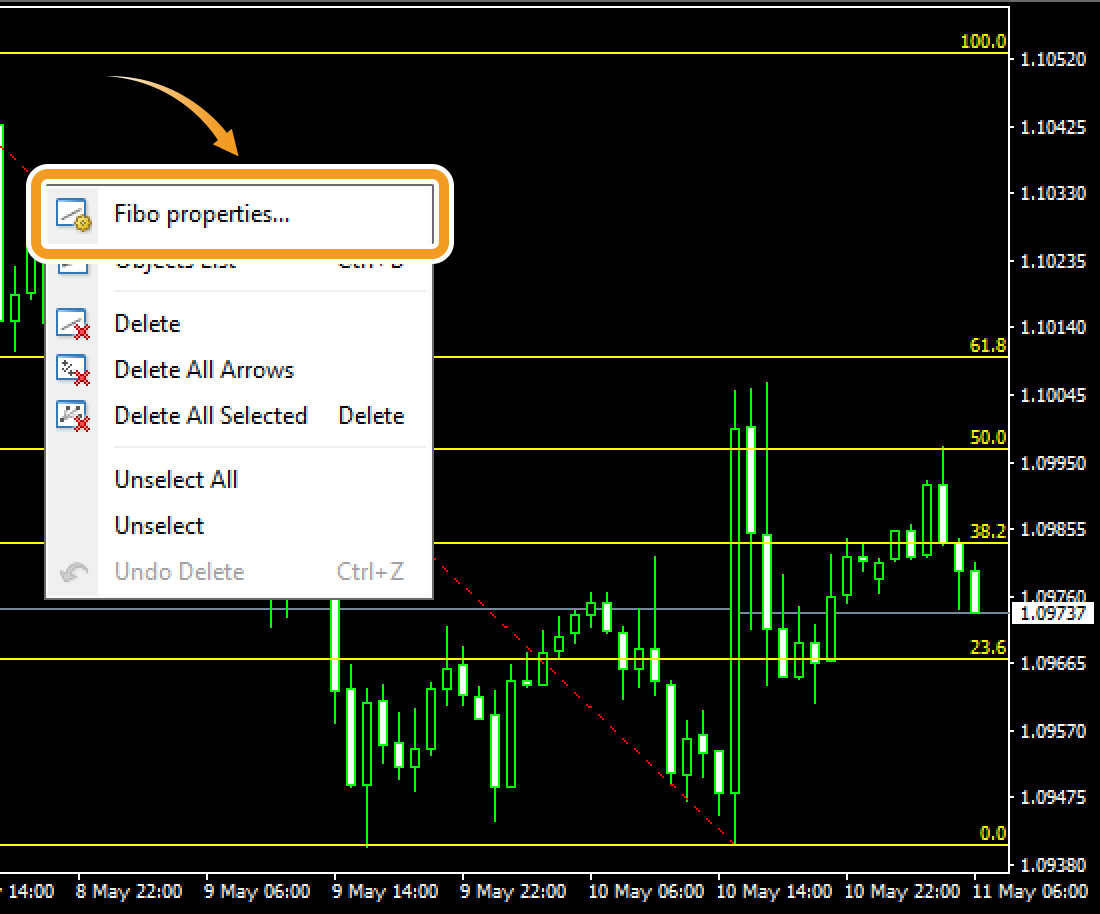## Step 5

On the "Properties" window, edit the parameters in the "Common", "Fibo Levels", "Parameters", and "Visualization" tabs and click "OK".

### "Common" tab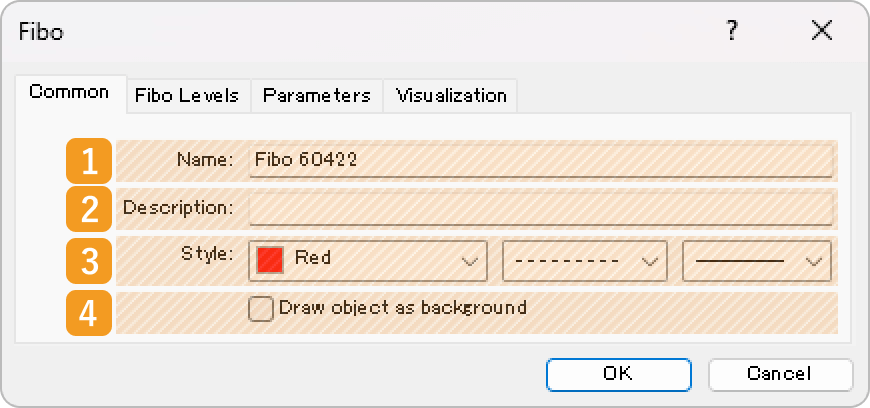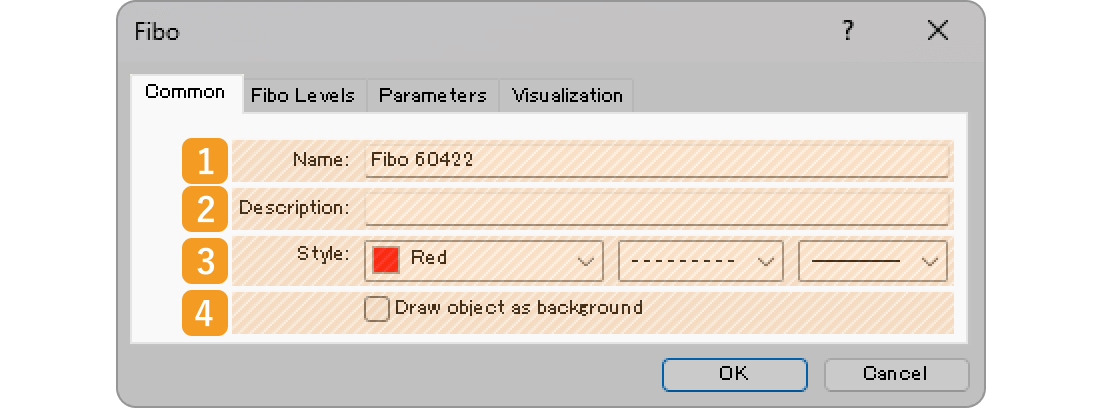Number 1 Item name Descriptions Name Name the Fibonacci retracement. Description The description can be displayed on the chart when hovering the pointer over the Fibonacci retracement. Style Set the color, line type, and line thickness for the Fibonacci retracement. Draw object as background Check this box if you want to place the Fibonacci retracement behind the chart.

### "Fibo Levels" tab

The Fibonacci retracement uses the Fibonacci ratio and draws lines at the 0%, 23.6%, 38.2%, 50%, 61.8%, 100%, 161.8%, 261.8%, and 423.6% marks. If you wish to change these numbers, double-click the number and enter your own value. If you wish to add a line, click "Add" and set the level and description.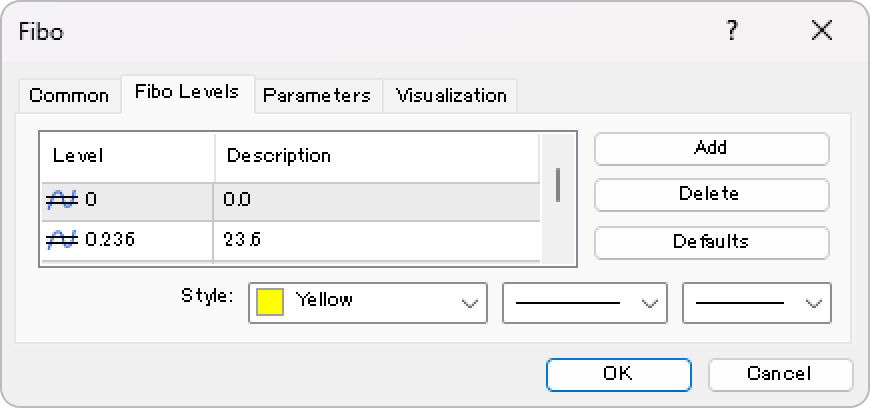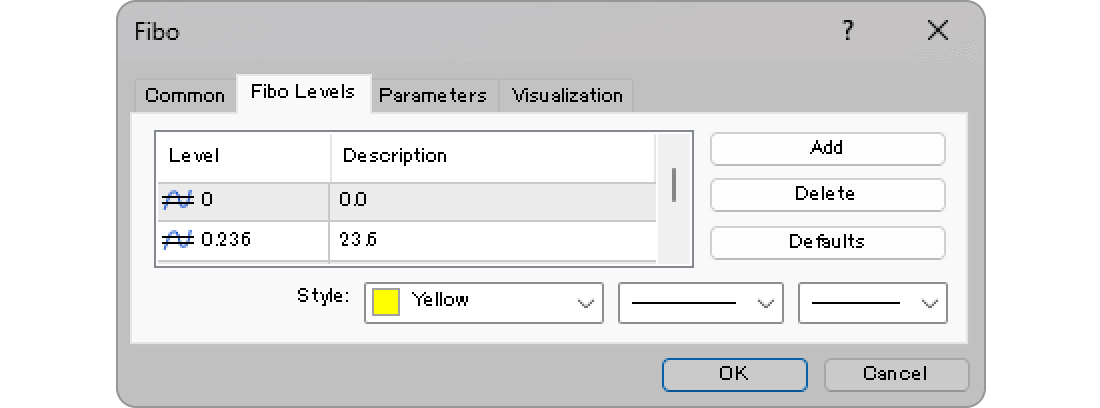### "Parameters" tab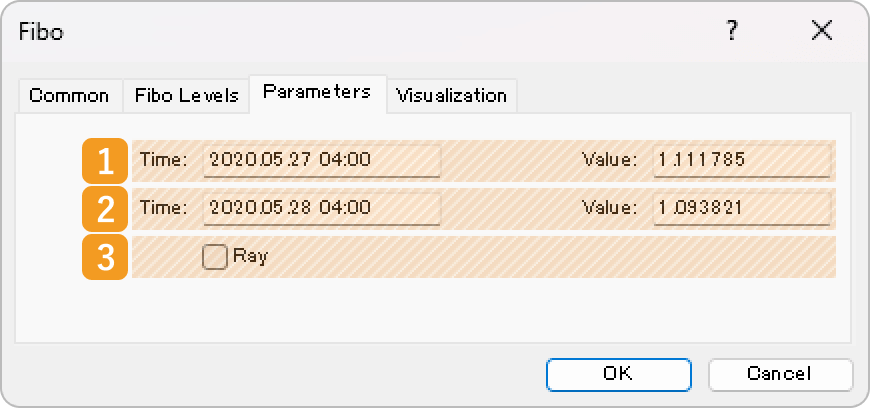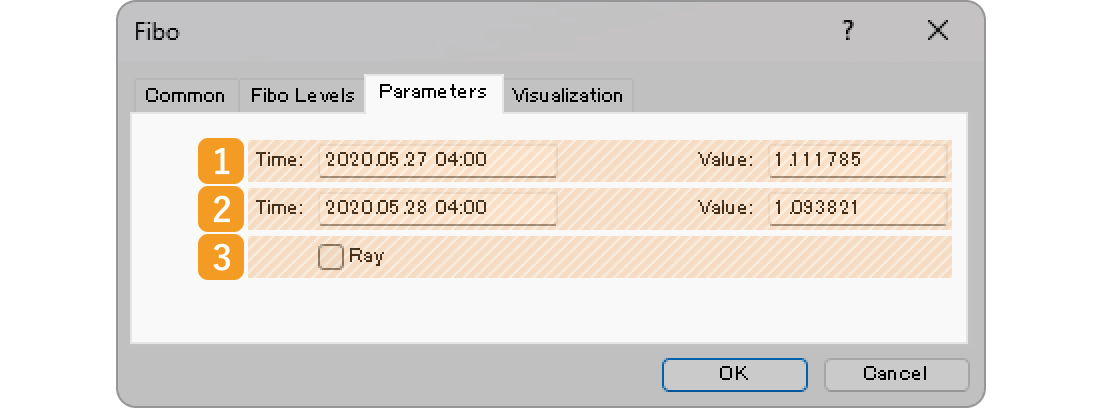Number 1 Item name Descriptions Starting point position Specify the starting point position by date and price. End point position Specify the end point position by date and price. Ray Check this box to extend the Fibonacci retracement to the right.What is "Ray"?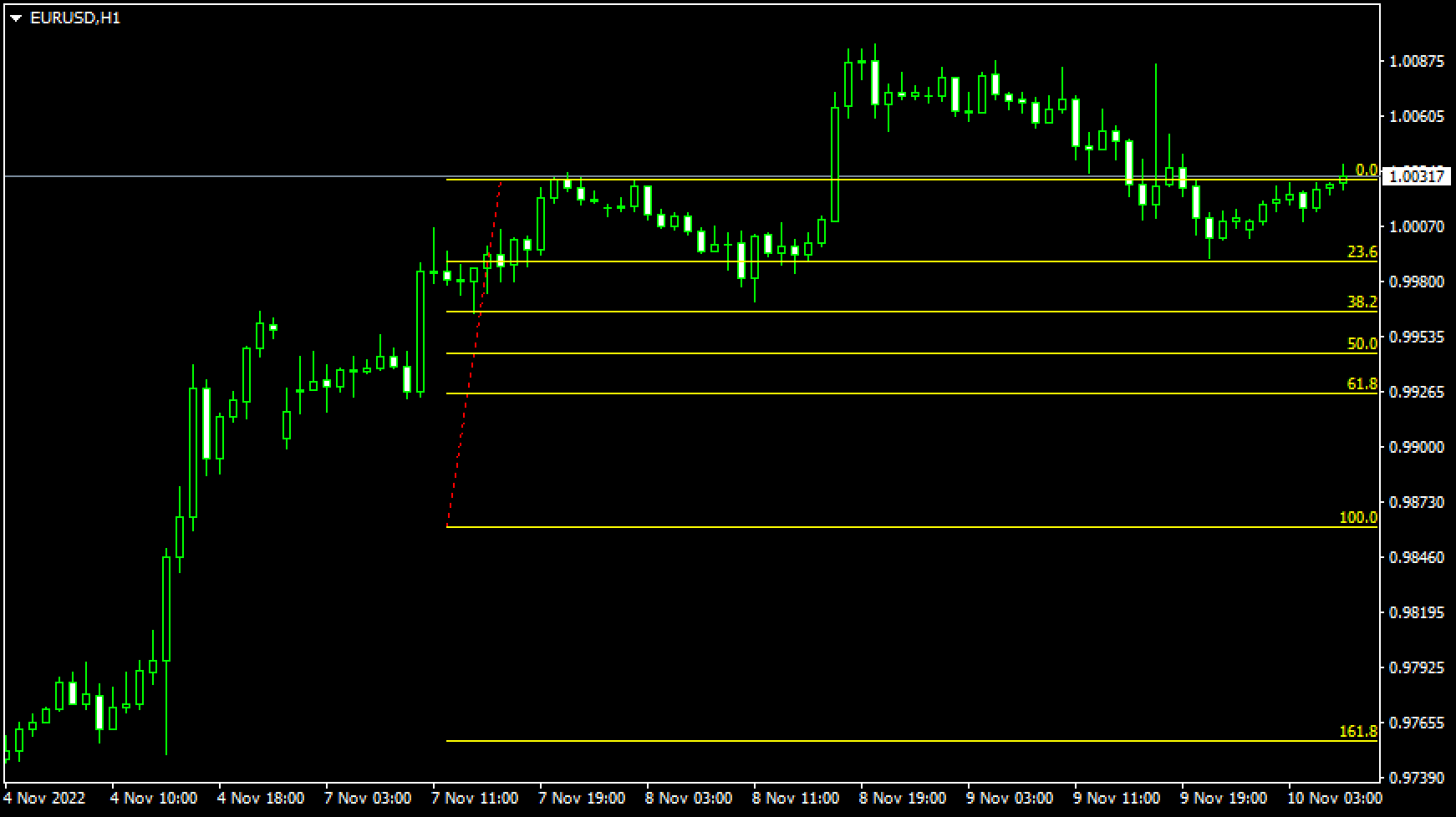If you check the "Ray" box, the line will be extended beyond its end point. This makes it easier to analyze, as the line will be extended into the future, based on the price movement between the starting point and the end point at a certain point in time.

### "Visualization" tab

On the "Visualization" tab, you can specify the timeframes to use the Fibonacci retracement with.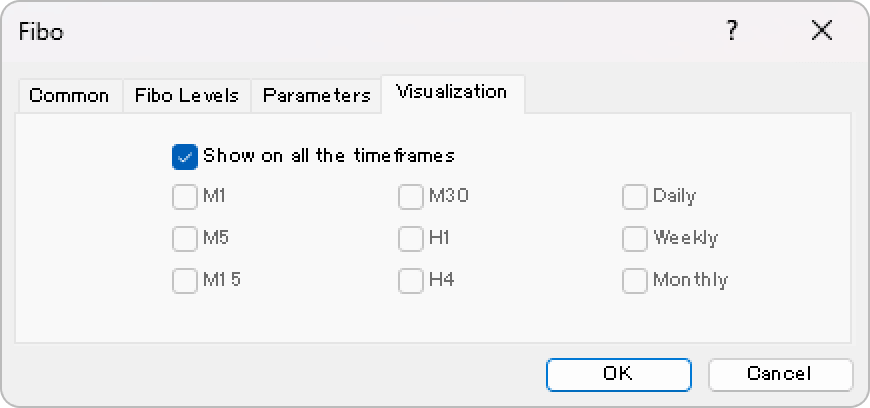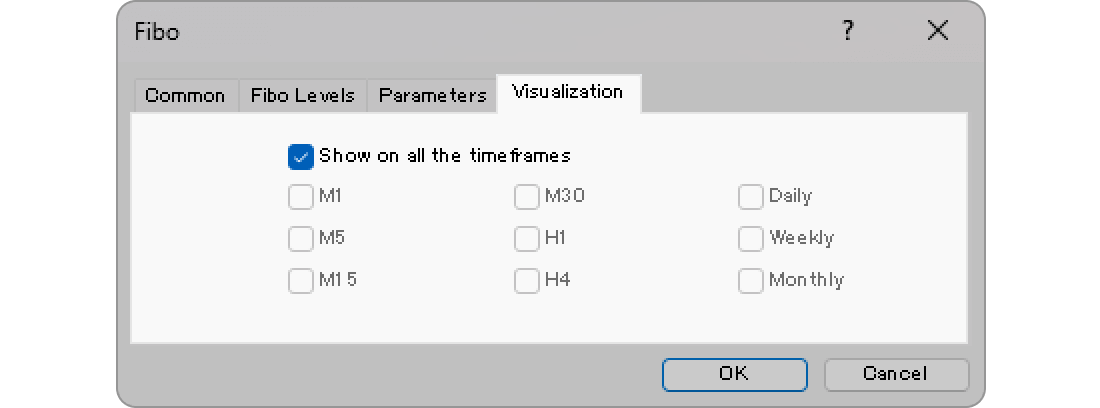## Step 1

Fibonacci tools are available from the menu or the Toolbar.

### Draw Fibonacci retracement from the menu

Click "Insert" in the menu. Hover the pointer over "Objects" > "Fibonacci" and select "Fibonacci Retracement".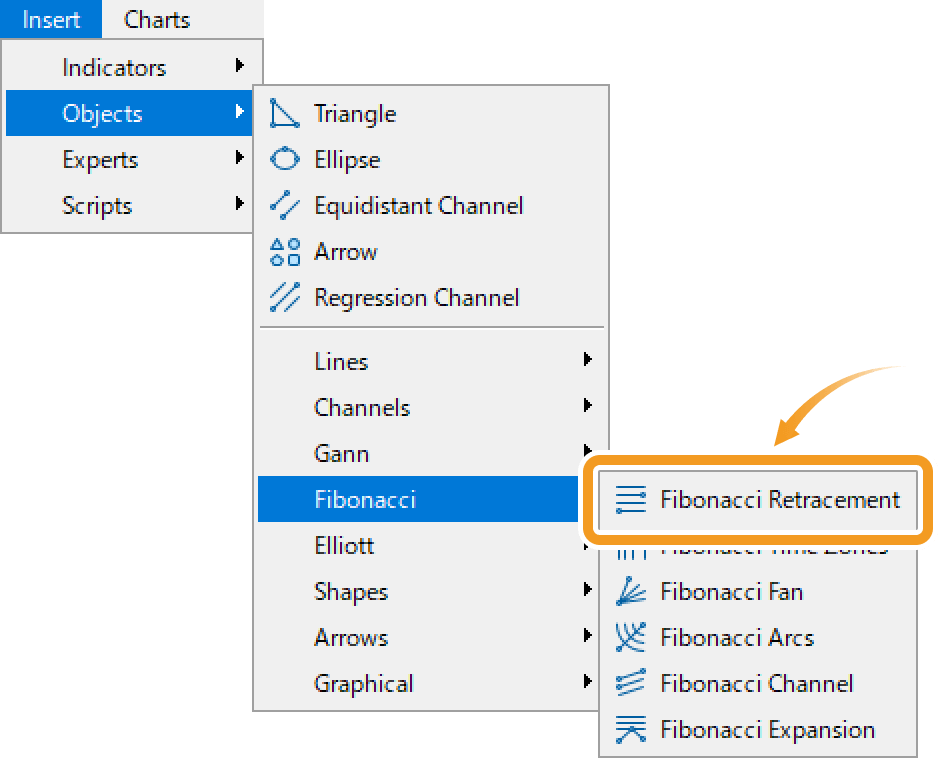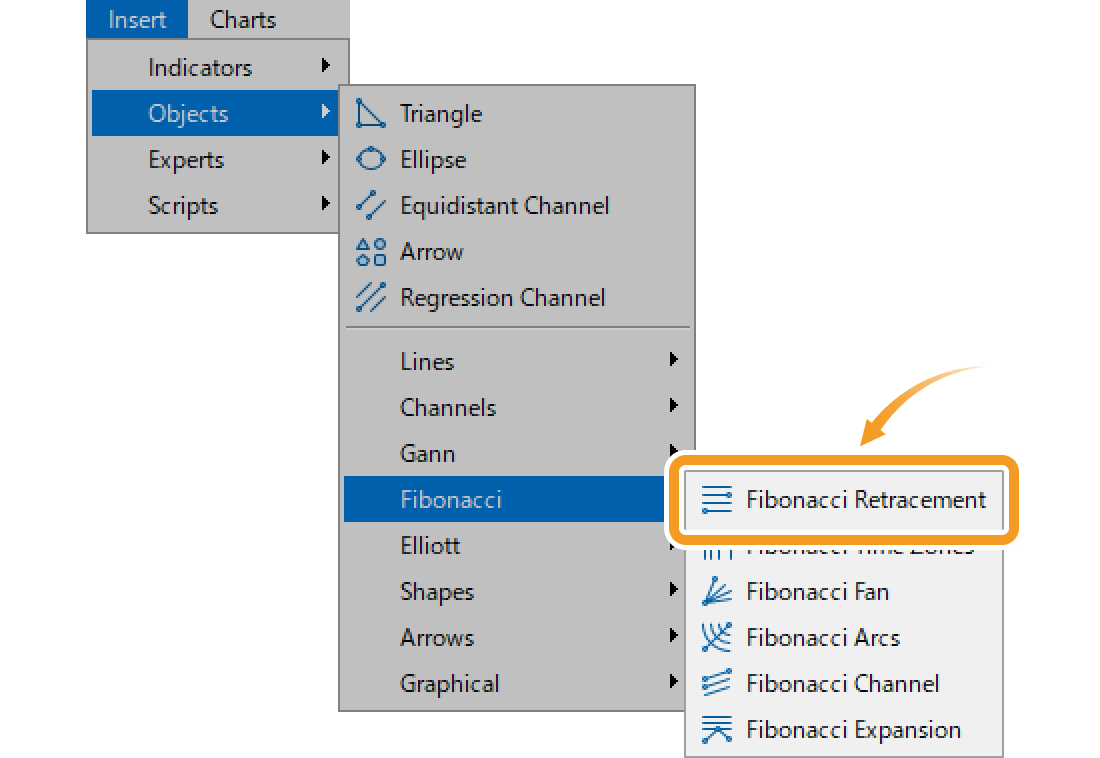### Draw Fibonacci retracement from the toolbar

Click the "Draw Fibonacci retracement" button in the toolbar.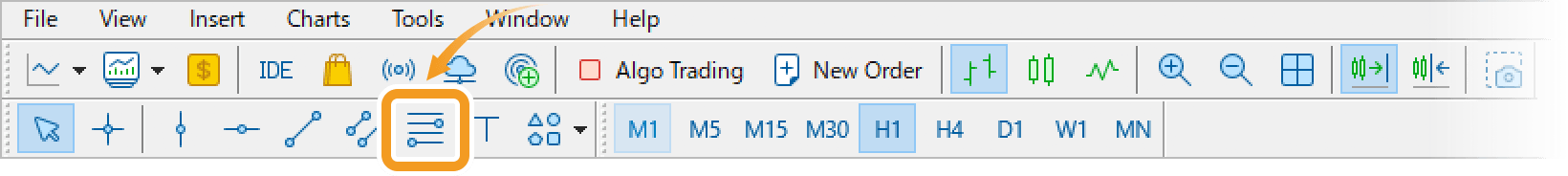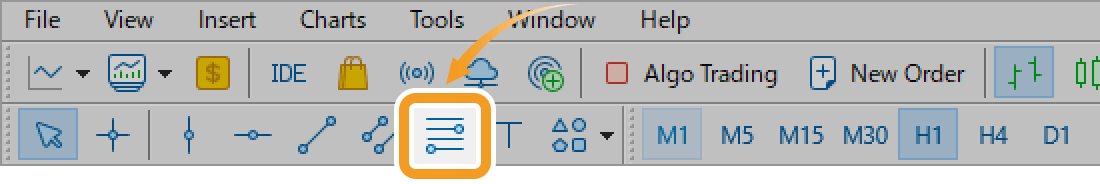1. Fibonacci retracement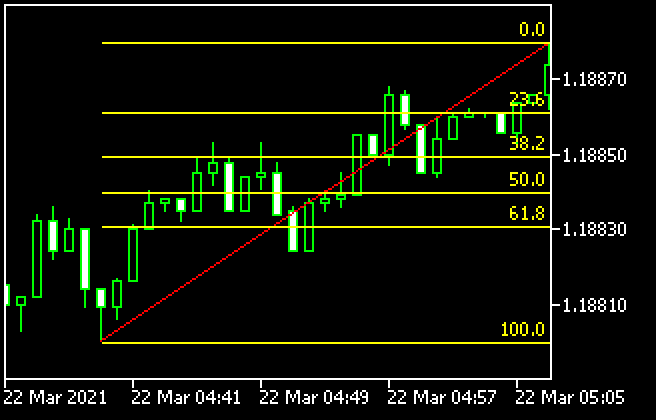Fibonacci retracement is a technical indicator that determines the temporary retrace price. It uses the Fibonacci ratio on the gap between the high and low to determine the dip/rally.

2. Fibonacci time zones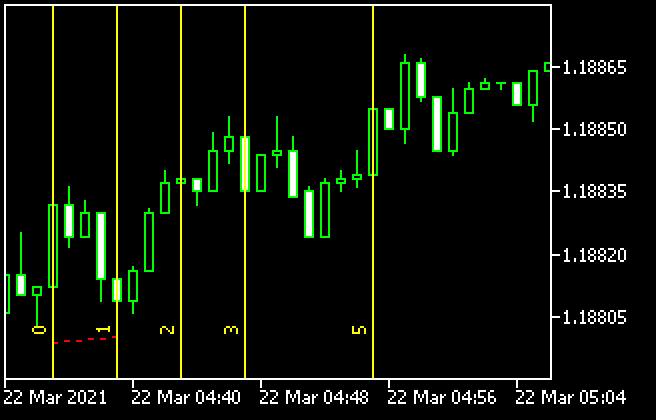Fibonacci time zones draw vertical Fibonacci lines relative to the time axis. Use these as reference points to determine when the trend will change.

3. Fibonacci fan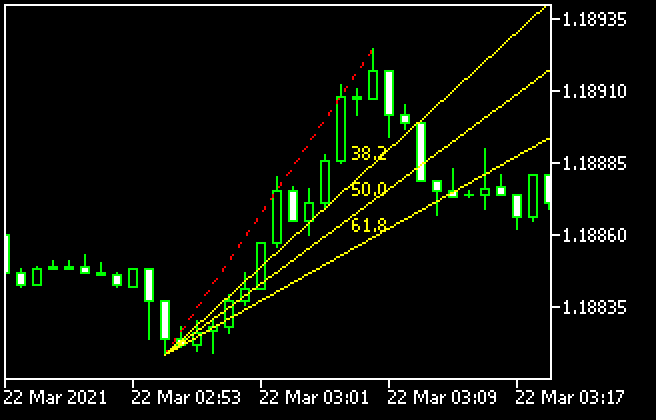Fibonacci fan draws 3 separate trendlines distanced from a starting point based on the Fibonacci ratio when you select the starting and end points of a wave. Use these lines as reference points to determine the support line and resistance line.

1. Fibonacci arcs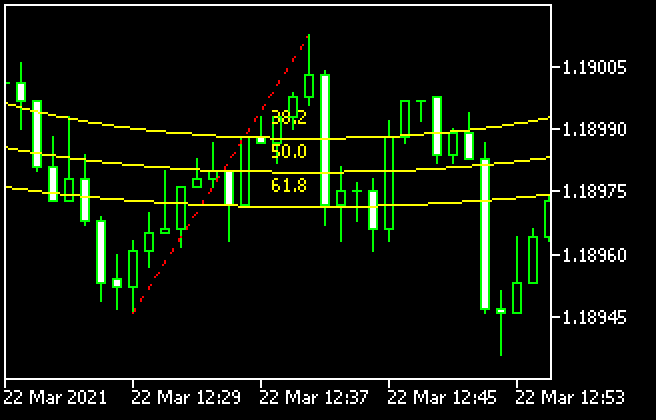Fibonacci arc is a technical indicator that utilizes the Fibonacci ratio on the price gap and time. Connect the high and low and it will draw 3 arcs relative to the end point using the Fibonacci ratio. Use these lines as reference points to determine the support line and resistance line.

2. Fibonacci channel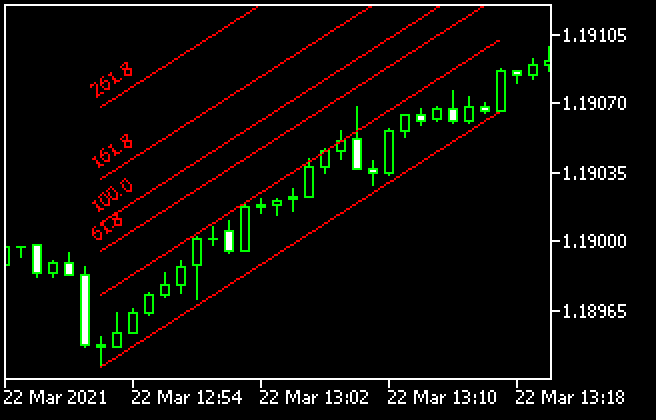Fibonacci channel uses the trendline and creates parallel channel lines using the Fibonacci ratio. Use these lines as reference points to determine the break points and when the trend will change.

3. Fibonacci expansion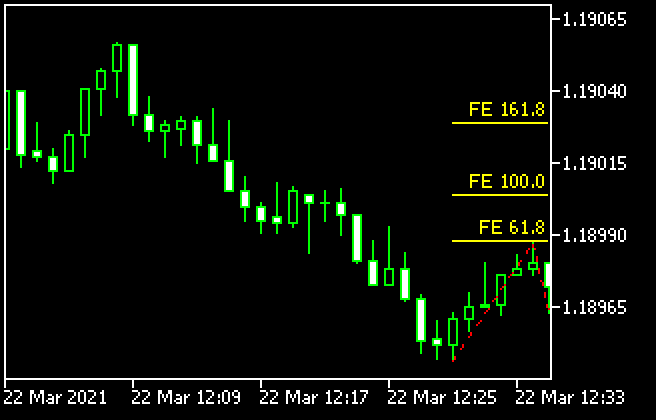Fibonacci expansion is a technical indicator that determines how long a trend will last. Connect the starting point of the trend and the dip/rally points and it will draw horizontal lines using the Fibonacci ratio. Use these as reference points to determine how far the market will expand.

## Step 2

Click the starting point on the chart and drag it to the end point to create the Fibonacci retracement.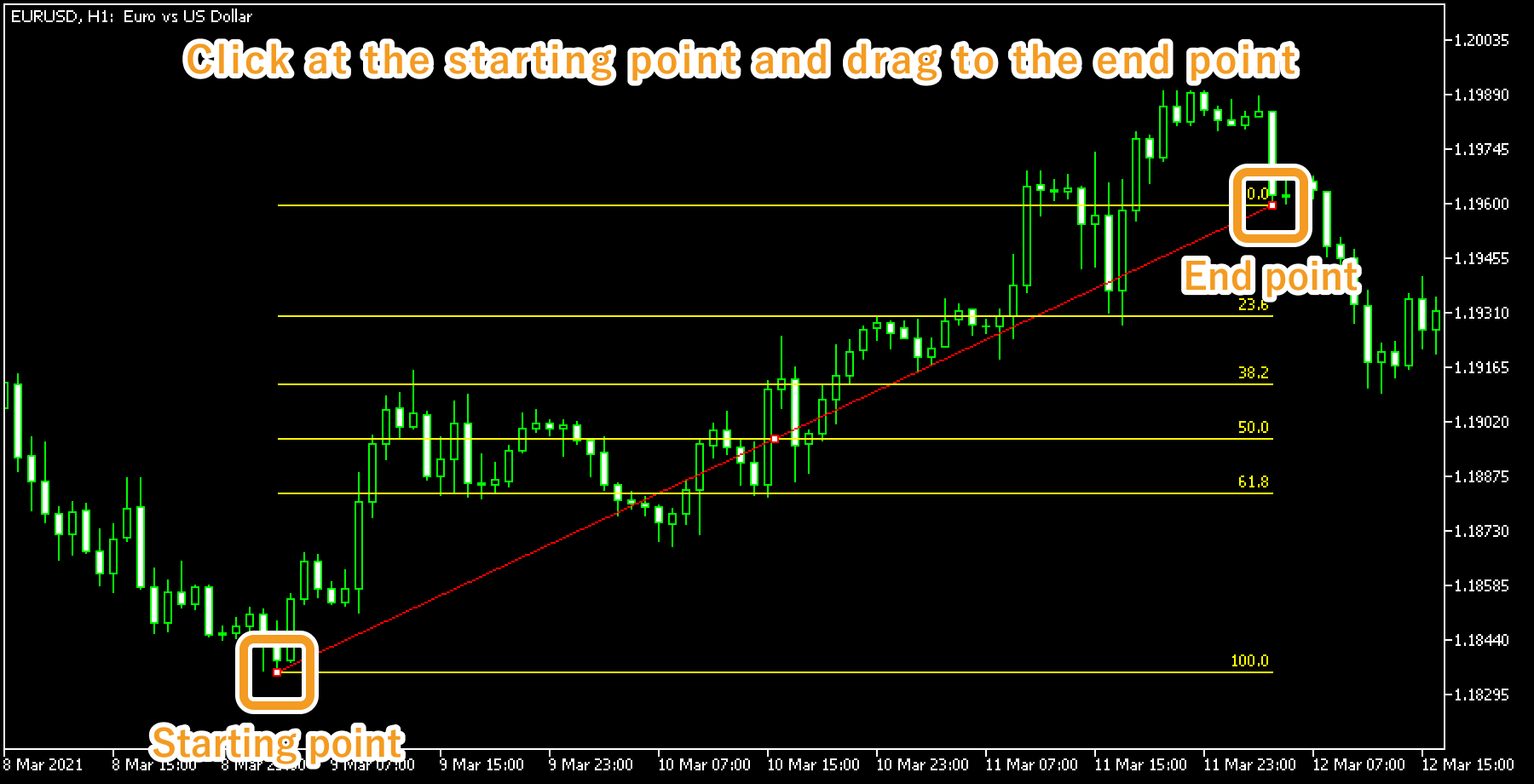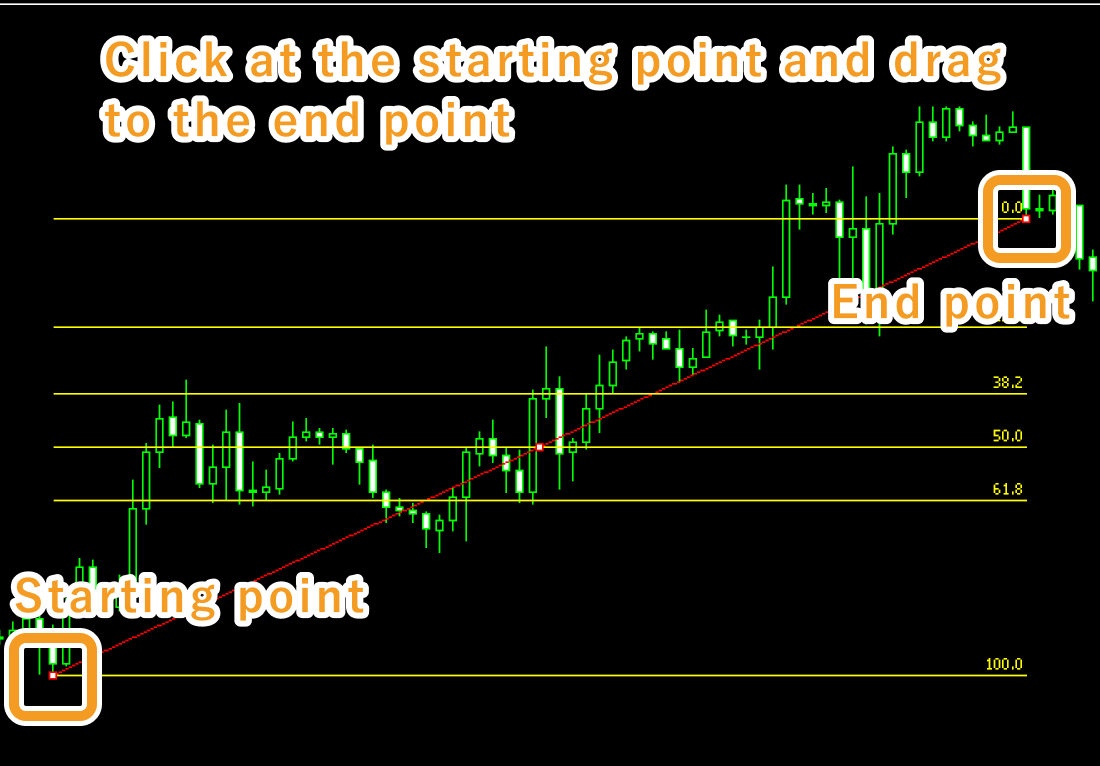## Step 3

If you want to adjust the position of the Fibonacci retracement, make sure the white dots are being displayed on the Fibonacci retracement and move each dot as necessary. If you cannot see the white dots, double-click near the Fibonacci retracement to display the white dots.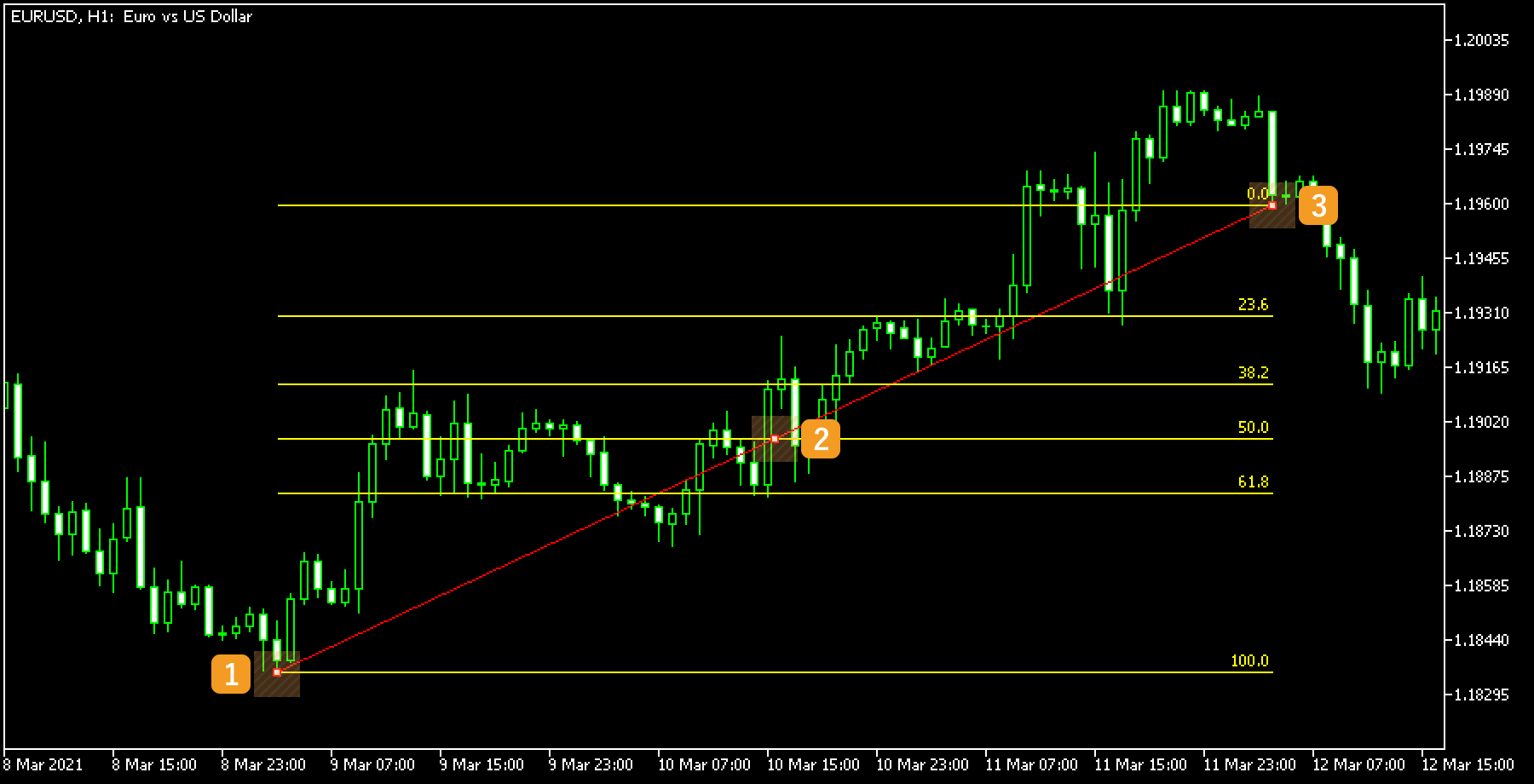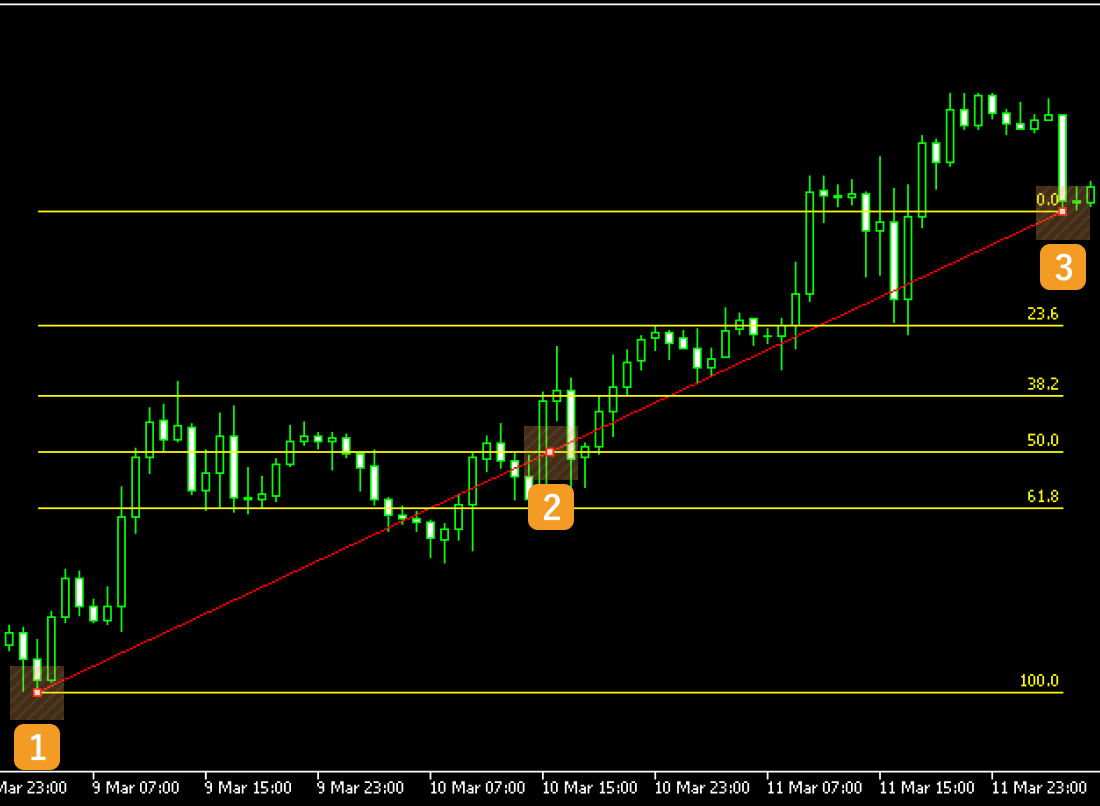Number Descriptions Change the starting point of the Fibonacci retracement. Change the position of the Fibonacci retracement. Change the end point of the Fibonacci retracement.Values displayed during drawing Fibonacci retracementv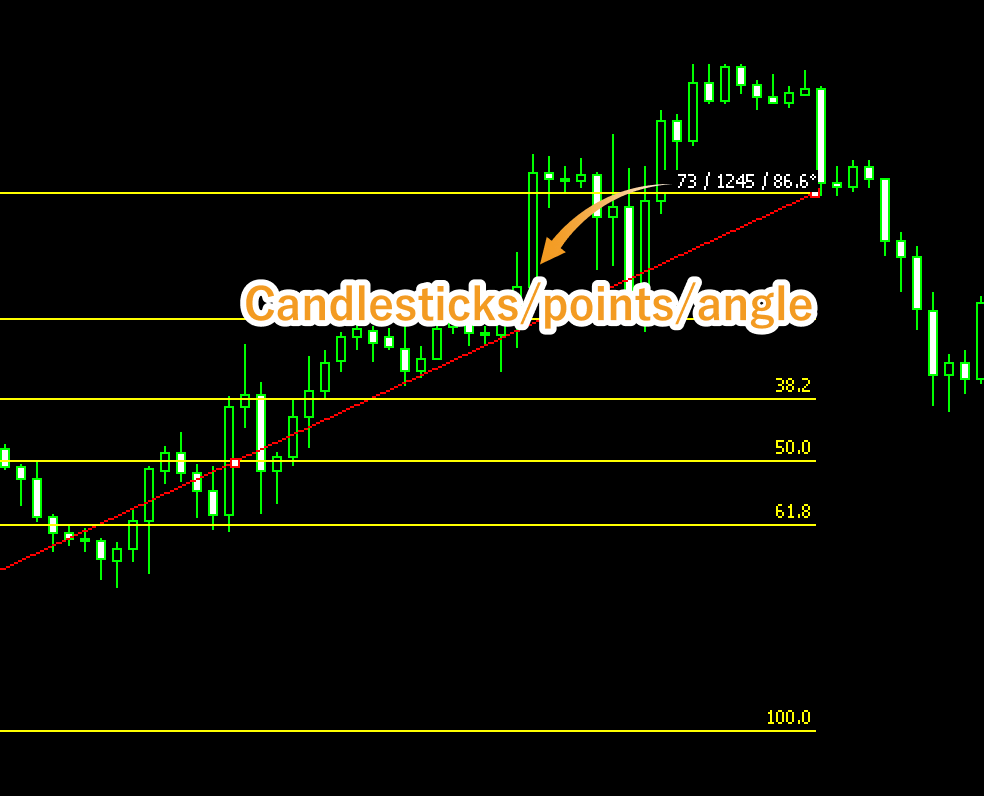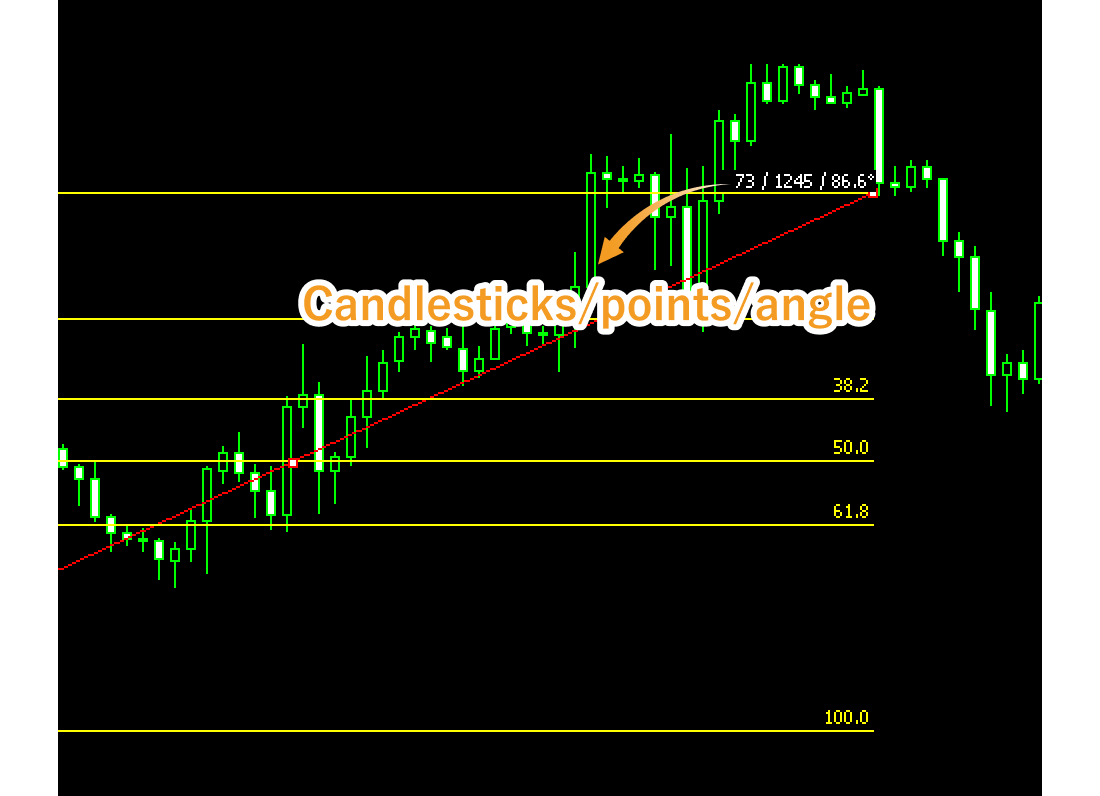Values displayed when dragging indicate the numbers of candlesticks and points from the starting point to the end point.

## Step 4

To modify the Fibonacci retracement settings, right-click near the Fibonacci retracement and select "Properties of (Fibonacci name)".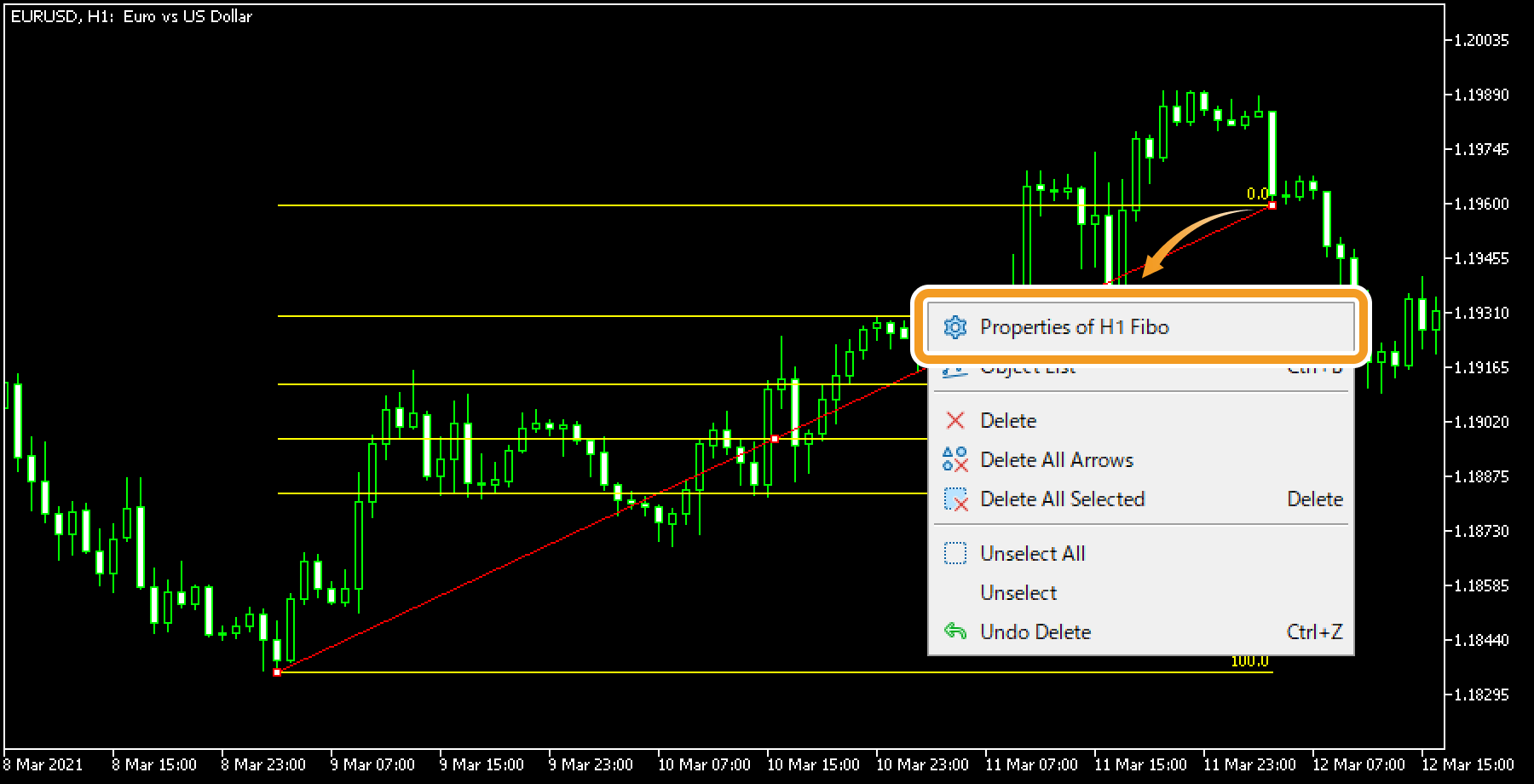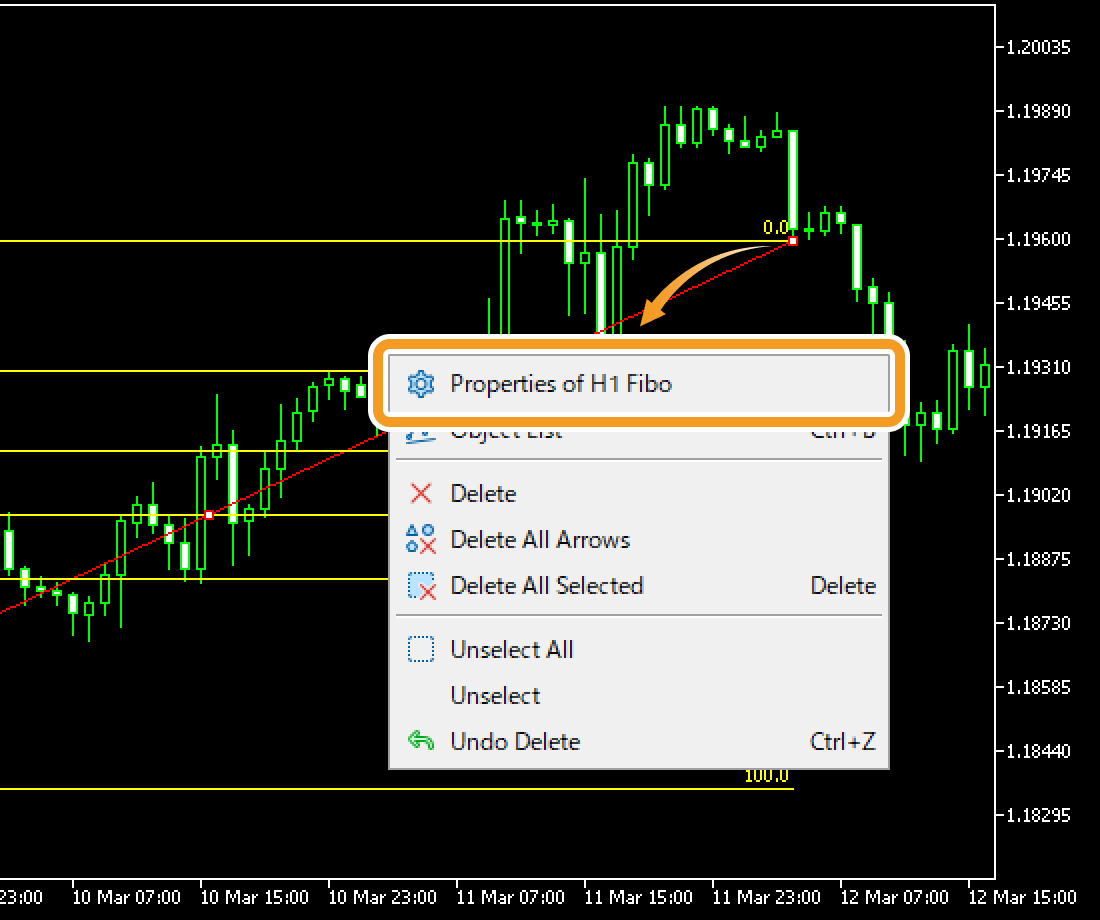## Step 5

On the "Properties" window, edit the parameters in the "Common", "Levels", "Parameters", and "Visualization" tabs and click "OK".

### "Common" tab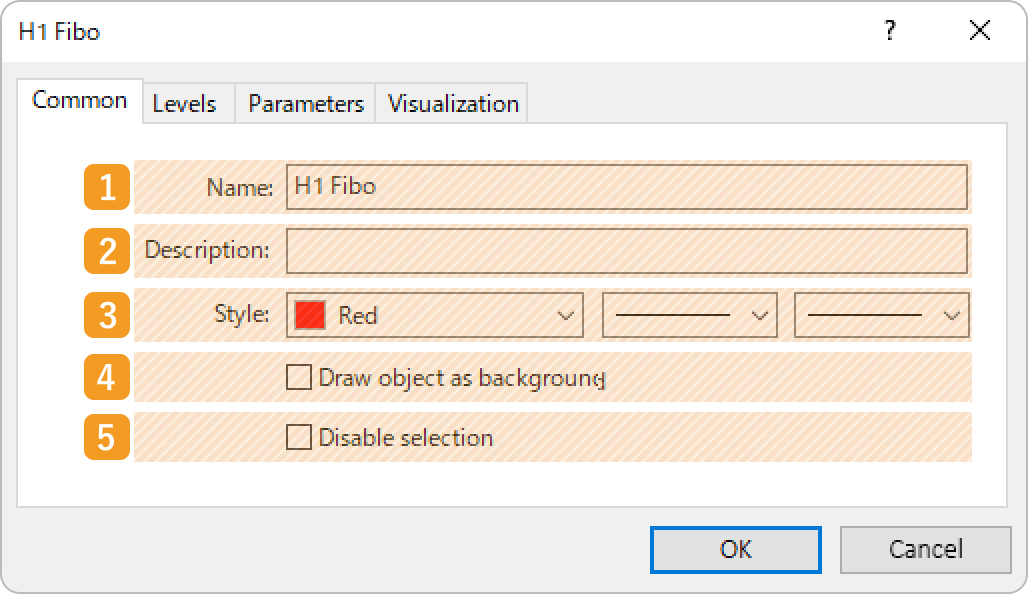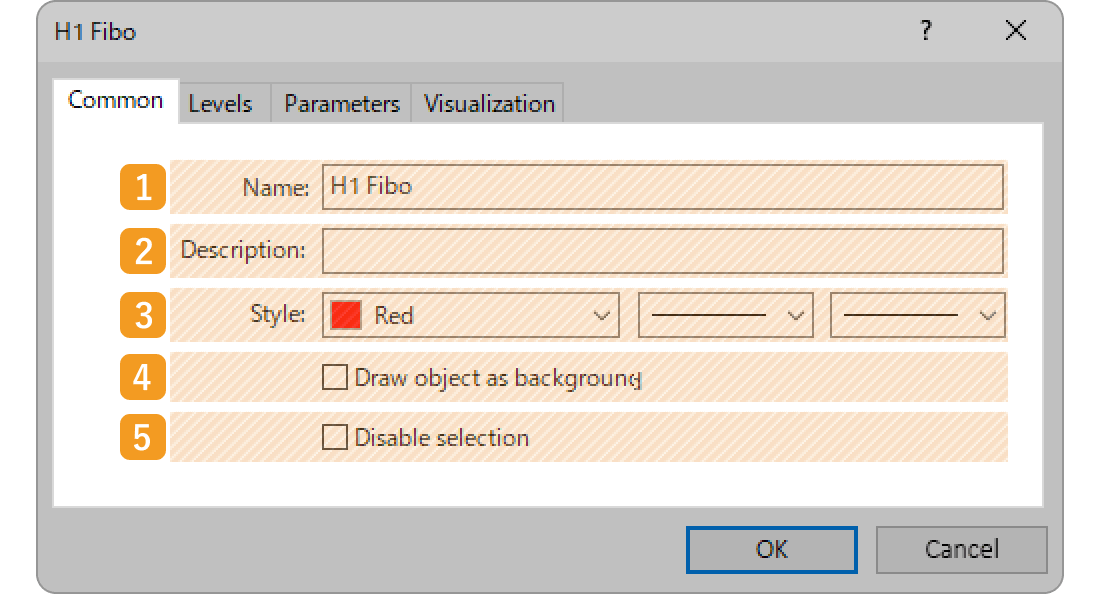Number 1 Item name Descriptions Name Name the Fibonacci retracement. Description The description of the Fibonacci retracement can be displayed on the chart. Related article: Show or hide items on chart Style Set the color, line type, and line thickness for the Fibonacci retracement. Draw object as background Check this box if you want to place the Fibonacci retracement behind the chart. Disable selection Check this box if you want to disable the editing of the Fibonacci retracement.

### "Levels" tab

The Fibonacci retracement uses the Fibonacci ratio and draws lines at the 61.8%, 100%, 161.8%, 261.8%, and 423.6% marks. If you wish to change these numbers, double-click the number and enter your own value. If you wish to add a line, click "Add" and adjust the value/settings.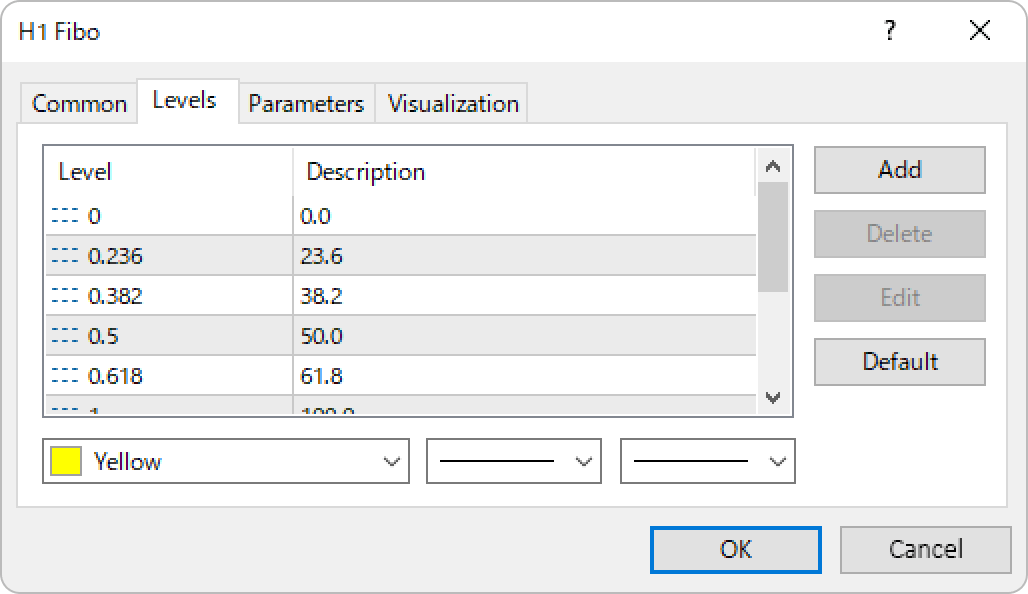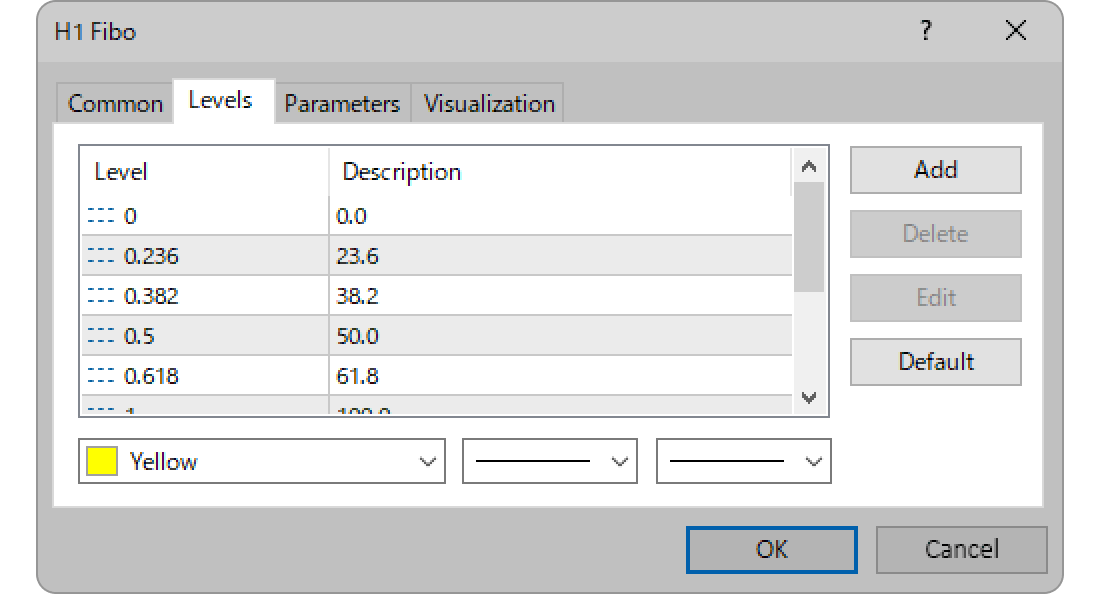### "Parameters" tab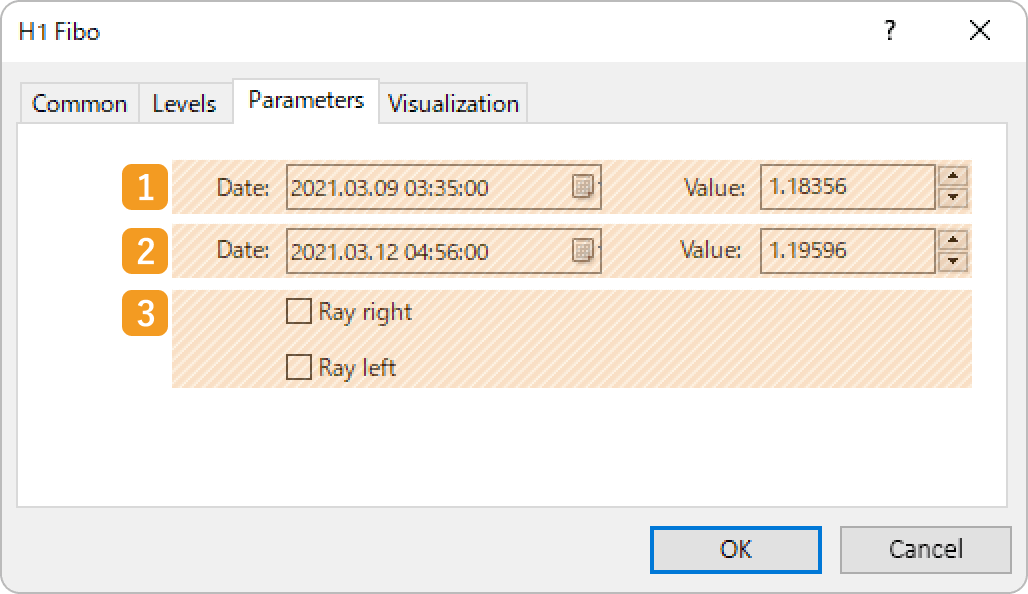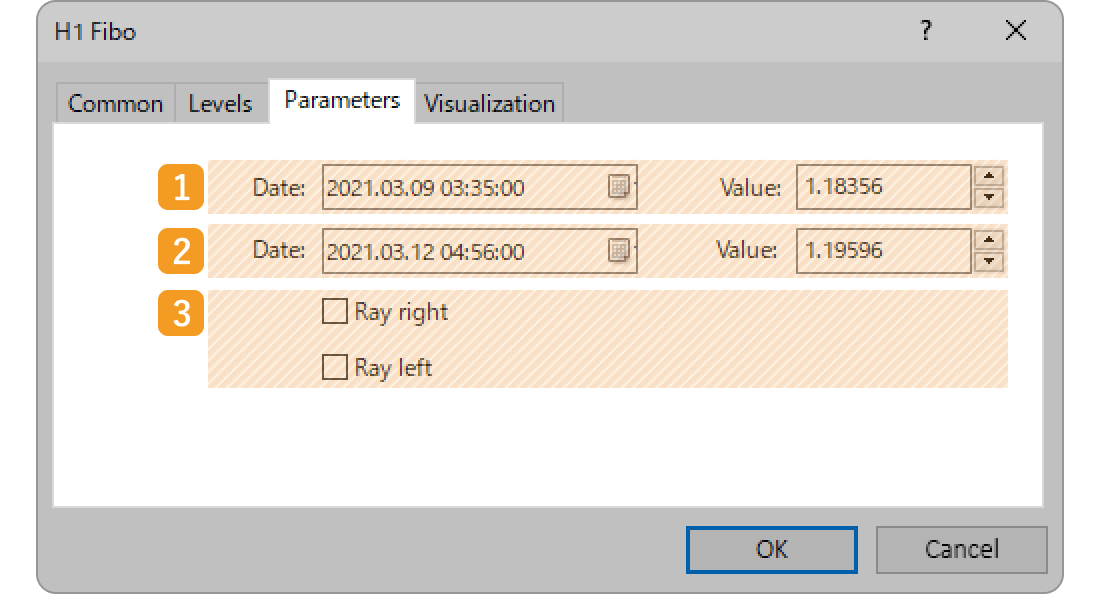Number 1 Item name Descriptions Starting point position Specify the starting point position by date and price. End point position Specify the end point position by date and price. Ray Check each box to extend the Fibonacci retracement to the left and right.

#### When "Ray right" is checked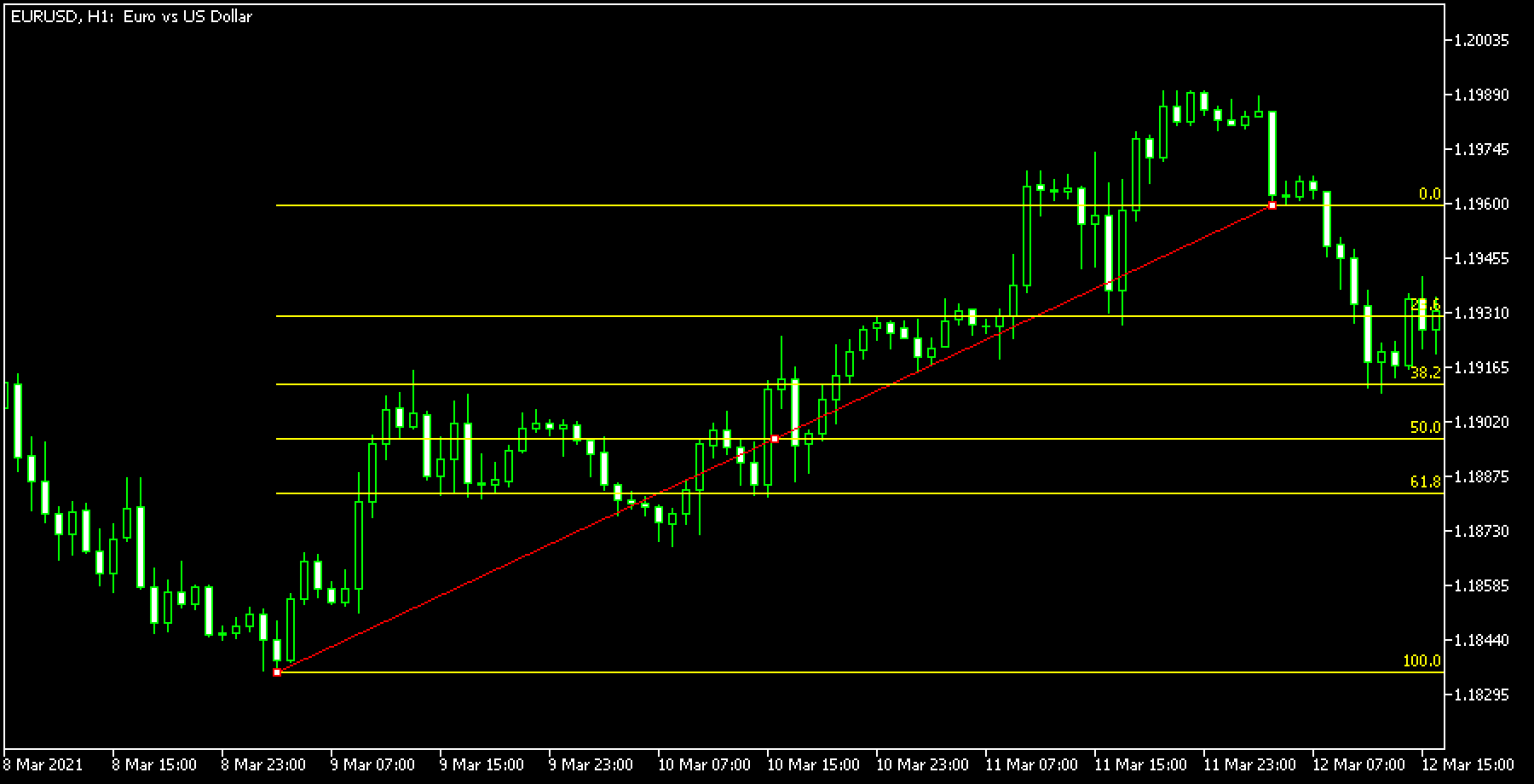#### When "Ray left" is checked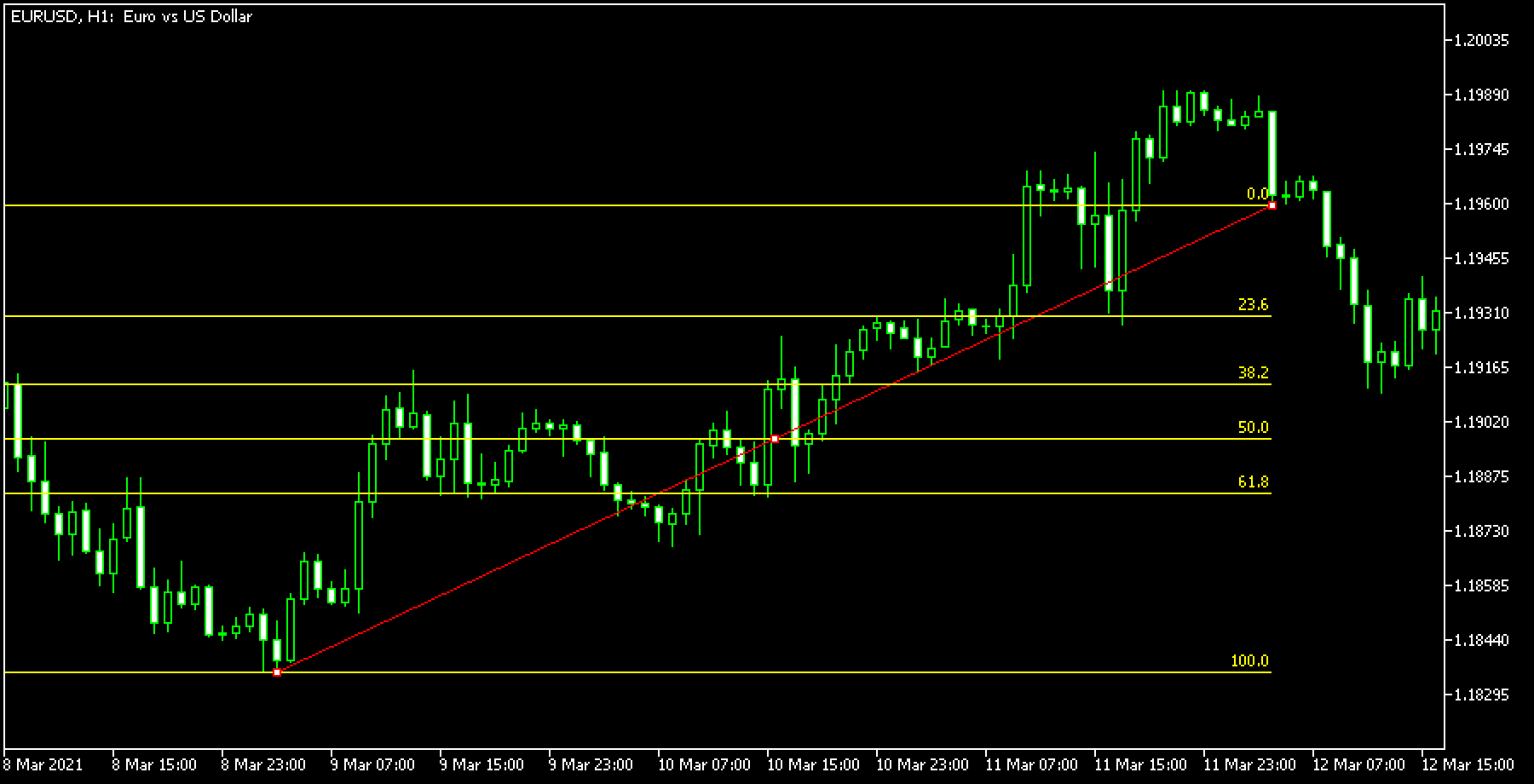What is "Ray"?

If you check the "Ray" box, the line will be extended beyond its end point. This makes it easier to analyze, as the line will be extended into the future, based on the price movement between the starting point and the end point at a certain point in time.### "Visualization" tab

On the "Visualization" tab, you can specify the timeframes to use the Fibonacci retracement with.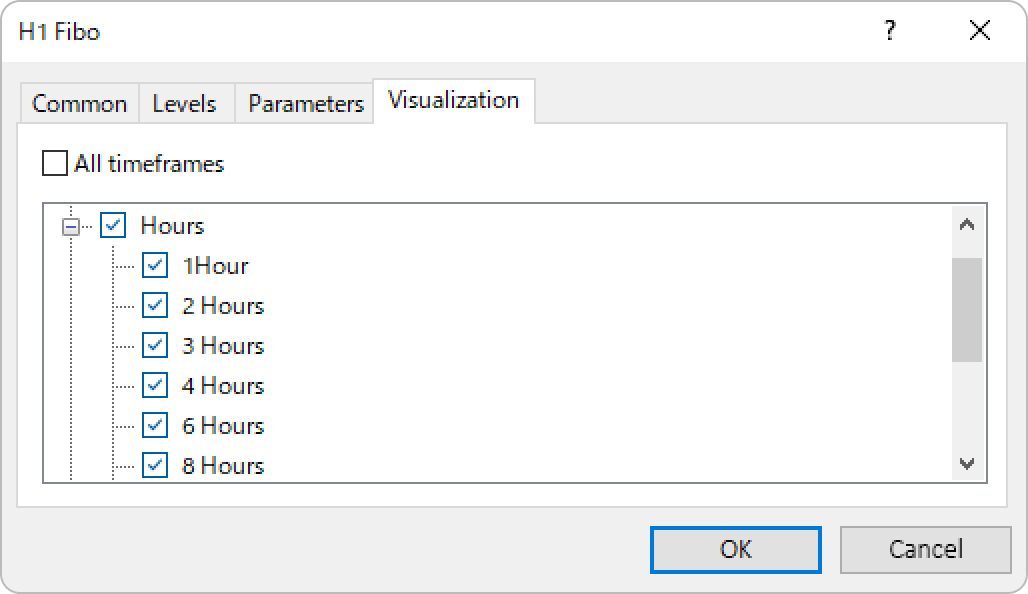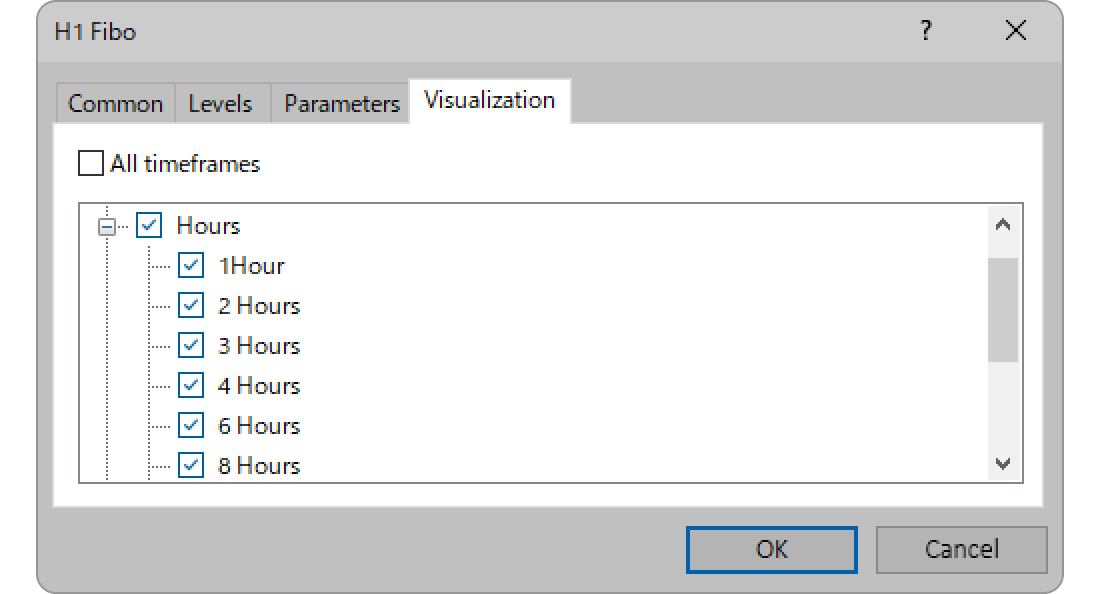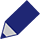Created

: 2022.09.09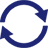Last updated

: 2023.06.16

Writer

Takayoshi Nakamaru

Manual production consultant, MBANakamaru is a manual production consultant at FINTECS, a company that specializes in creating manuals for their clients.
With a wide range of experience from fintech to entertainment, he presents what user-friendly manuals should be like.
He works with numerous large corporations as an external manual production project manager.

http://www.fintecs.co.jp/profile/

Supervisor

Akira TakagiAfter graduating from Computer Science at the Uninove, Brazil, in 2014, he has worked on various systems development projects.
He participated as a developer in the launch of forex services in 2019. Since then, he has also been involved in the development of MetaTrader plugins and APIs. He is certified by MetaQuotes as a MetaTrader5 Administrator and active in consulting and advisory as well.

We are also looking for writers with extensive experience in forex and crypto to join us.

Disclaimer: